# Machine Learning Summary (Fundamental)San Francisco Exploratorium

“One learning algorithm” hypothesizes that human intelligence may be due to one learning algorithm. Unfortunately, we are not there yet. Machine Learning (ML) starts from many disciplines and there are a lot of concepts to learn. Sometimes these concepts are explained with too much abstraction or on the contrary, too little substance. In this series, we summarize the fundamental and study the major ML algorithms. For this article, some complex concepts include Bayes inference, Expectation-maximization Algorithm, Naïve Bayes Theorem, Hidden Markov Model, PCA, Markov Chain Monte Carlo, Metropolis-Hastings algorithm, Variational inference, Moore-Penrose Pseudoinverse, Statistical Significance, etc … will be covered. However, to shorten the length of the article, we assume that you have basic exposure to ML. If you have problems following the material, I suggest the section at the end for some ML learning resources. The articles are long. Feel free to skip some sections based on your level of knowledge and interests, in particular in the beginning.

### Information theory

Let’s introduce some concepts in information theory. The Shannon information content is the amount of information gain when an event x occurs. Mathematically, it is defined as

For example, if a coin is biased and shows heads all the time, the events are predictable and we know nothing new. Therefore, the information content is zero. If the coin is unbiased, the probability distribution is uniform and what exactly happened is most unpredictable. The information content is the highest. Take a second to digest it, it may be at odds with your concept of information. In particular, uniform distribution leads to the highest content.

In computer science, we view the information content as the number of bits to encode the information most efficiently. Utilizing the event frequency, the optimal encoding scheme will be`0b0` for head and `0b1`for tail in a fair coin (or vice versa). For our discussion here, our log is in base 2. Therefore, the information content for head or tail in flipping a fair coin is -log₂(½) = 1. i.e. we gain 1-bit of information when we flip the coin once.

X is called a random variable if it holds a value for the outcome of a random process (a stochastic process), e.g. the face value in rolling dice. Entropy H measures the expected information content of a random variable according to its data distribution. To calculate the value, we sum up all information content with a weight equals to its occurrence probability.

For example, in flipping a fair coin, P(X=head)=½ and P(X=tail)=½. Its entropy equals

The average content is one bit. We use an average of one bit to encode an event.

Cross-entropy

Conceptually, a random variable is generated (created) from a data distribution, says P. For a fair dice, P(x=head)=P(x=tail). Cross-entropy H(P, Q) measures the number of bits to encode X using an encoding scheme targeted for distribution Q.

In ML, we want our predictions to match the ground truth which P is the ground truth and Q is the model prediction. For example,

KL-divergence

In ML, random variables are described with distributions. KL-divergence is often used to measure the difference between two distributions P and Q — one for the ground truth and the other for the predictions made by our model.

Cross-entropy, entropy and KL Divergence are related as:

It measures the inefficiency of representing P with encoding scheme Q. In ML, we use KL-divergence to measure the difference between two probability distribution. However, entropy is not a function of the model parameters. Therefore, optimizing KL divergence w.r.t. model parameters is the same as optimizing cross-entropy.

We can optimize the cross-entropy or the KL-divergence in creating a model.

It takes extra-bits to encode information with a sub-optimal scheme. Therefore, KL-divergence is always greater or equal to zero. There are a few properties of the KL-divergence that need to be aware of.

KL(p, q) ≥ 0
KL(p, p)=0, and
KL(p, q) ≠KL(q, p) (non-symmetry)

Information Gain

Mutual information (or Information gain) I(X; Y) is the information obtained on the random variable X when Y is observed. The mathematical definition can be confusing.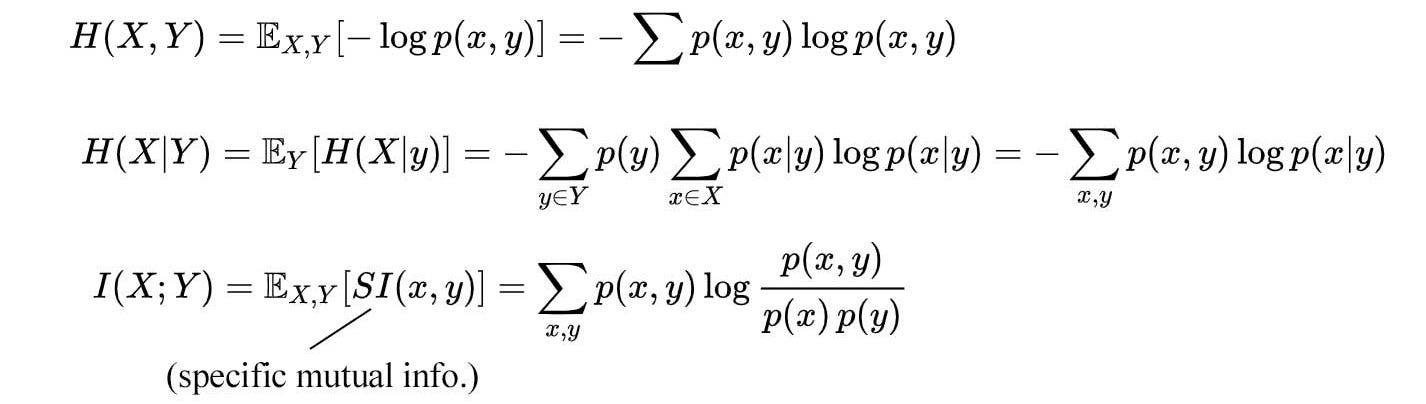Modified from source

But it can be understood better with entropy

Intuitively, how much information do we gain by knowing X? For example, if we know the label (Y) of an object, we gain a lot of information about its raw image (X). We should not mistake its picture with other objects. Therefore the information gain I(X;Y) is high. This concept can be further elaborated with the relationship between the entropy and the mutual information described below.

If random variable X and Y are unrelated, their intersection is empty and the mutual information is zero. If random variables X and Y is the same, H(X)=H(Y)=I(X;Y), knowing Y is the same as knowing X. In a decision tree, we choose a condition to maximize I — a condition that gains the maximum information by separating data according to the branching condition. In another example, InfoGAN maximizes the mutual information between the generated image G and its intended label c. This encourages the GAN model to generate images related to its intended label.

Conditional Entropy

The conditional entropy H(Y|X) is the weighted entropy of Y given X=x. (E.g. H(car price | color = red).

### Probability

Probability mass function is the probability distribution for discrete random variables. For example,

Probability density function (PDF) is the probability distribution for continuous random variables with lower case notation p(x). In principle, p(x=xᵢ)=0. The chance of having a weight exactly equals 10.0000000000 lb should be zero. Instead, we calculate the probability between two values by integrating PDF over its range (say between 9 and 10)

Cumulative distribution function (CDF)

CDF calculates p(X≤x).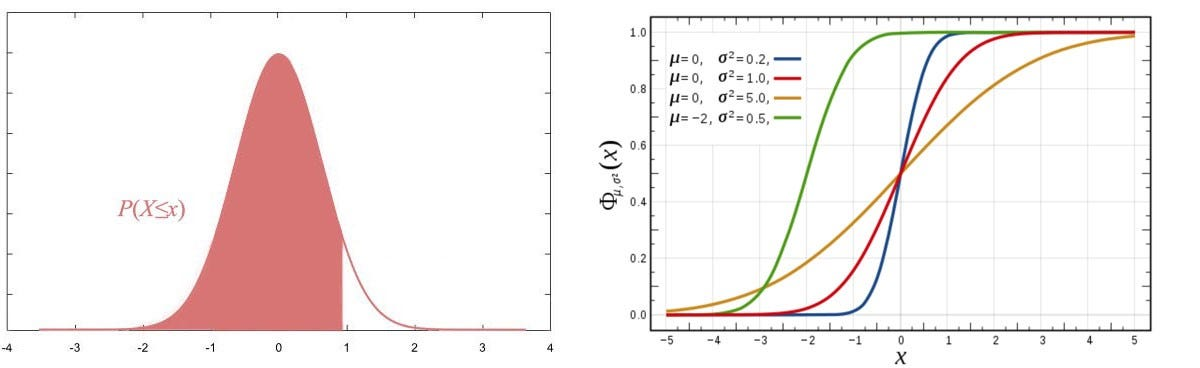CDF on the right (The CDF image source)

Conditional probability

Independence

Two random variables A and B are independent if

Marginal probability

A joint probability P(x, y) may be much easy to observe or to model compared to P(x). Sometimes, that is the observed data we have collected. The marginal probability P(x) is computed by summing (or integrating) the joint probability over y.

Chain rule

Bayes’ theorem

The equation looks simple but it is one of the most important equations in ML. So it is important to check out here to get familiar with the terminology. Researchers will explicitly use these terms in describing their concepts and make you miserable if you are not used to them.

Bayes’ Theorem can be formularized in different forms. We will use the following forms frequently.

Let’s say there is a genetic disease that only 0.1% of the population gets it. We develop a test with 99% accuracy for the positive and negative result. So, if you test positive, should you be overly worried. Intuition will say yes but in reality, the Bayes’ Theorem proves that there is only a 9% chance that you get the disease (proof). The intuition is not hard. Since the disease is so rare, most of the positive results from the lab come from false positives of people without the disease — a small portion of a large pie is still larger than a big portion of a very small pie.

Bayes inference v.s. Point estimate

In linear regression, we use a point estimate for our prediction. We predict a definite value based on the input features. For example, the price of the house can be calculated from the square footage and the number of rooms (price = a × square footage + b × # of rooms + c).

In Bayes inference, we apply Bayes’ Theorem in making a prediction. Both the prior and likelihood is a probability density function (PDF). The calculated posterior is also a PDF. With Bayes’ Theorem, we predict a distribution of values and our certainty on these values. The expected value of the PDF should match the point estimation of other accurate ML methods. As shown later, this gives us flexibility in combining a prior belief or opens the door for further mathematical manipulation using probability and distribution theory. For engineers, our calculations are very deterministic. So please take some extra time to digest this probability concept. We are working on a curve rather than a single point estimation.

Probabilistic models & non-probabilistic models

In general, models can be categorized as probabilistic models and non-probabilistic models. Probabilistic models use probability distribution to model a problem and to make predictions. Those models are usually built on MLE (Maximum likelihood estimation) or MAP (Maximum a posteriori estimation). For example, in linear regression, we optimize our model parameters w to maximize the likelihood of the observed data. In Bayes’ Theorem based solutions, we are interested in more than a point estimate. Instead, we are interested in estimating p(x), p(y|x) or p(model | observations). We answer questions like what are the different rates of the contractors and the corresponding probability.

Probabilistic models are about reasoning about uncertainty. We model the uncertainty in our data, our model and/or our answers. With Bayes’ Theorem, we explore all possible answers with the corresponding certainty. Of course, this may not be easy. If the analytical solution is intractable, we approximate the solution to reduce the problem complexity. Non-probabilistic models do not use distribution to model them. These models include clustering, SVM, decision trees, etc …

Advantages and disadvantage of Bayes’ Theorem

In some ML problems, finding P(y|x₁, x₂, x₃,  ) can be hard. With Bayes’ Theorem, we can compute this value by computing P(x₁, x₂, x₃, …|y) instead. In some problems, this may be easier because the collected sample may answer questions on P(x₁, x₂, x₃, …|y) or some of its variants better. As shown later in Naive Bayes’ Theorem, this probability can be further broken down into independent components and solved easily. Also, in early experiment stage, the evidence is weak since we have not collected enough samples yet. But if we have a solid prior belief, we can still make reasonable good predictions using Bayes inference. Don’t worry about the details now. More specific examples will come soon.

However, the equation for Bayes’ Theorem is deceptively simple.

The calculation in our disease example is very simple because the number of states is small. However, multiply the prior and the likelihood curve in high-dimensional space or continuous space is often intractable. The integral for the marginal probability is hard to compute also.

In practice, we can solve them by sampling or approximation. For example, we can model the prior and the likelihood with Gaussian Distributions. The posterior distribution will be Gaussian and easy to compute analytically.

In ML, we often use Bayes inference to find the model w using the posterior distribution.

The marginal probability in the denominator sums over w and is no longer a function of w. Hence, it is a constant w.r.t. w. Therefore, we can ignore. We can find the same optimal solution with or without the marginal probability.

Naive Bayes’ theorem

We can apply Naive Bayes’ theorem to solve classification problems. As explained before, Naive Bayes’ theorem helps us to solve P(y|x) when P(x|y) is easier to calculate. In particular, when x is composed of independent events, P(x₁, x₂, …|y) can be further simplified as P(x₁|y)P(x₂|y)… Therefore, P(y|x) can be computed as

Originally, the exponential combinations of x₁, x₂, …, xn makes it too hard to collect data to estimate P(y | x₁, x₂, …). After applying the Bayes’ Theorem, we can further break down the likelihood into independent events. This reduces the complexity of the model significantly and makes the problem solvable. Let’s use Naive Bayes’ theorem to detect spam emails. People often mark their spam emails and these spam emails tend to use certain vocabulary frequently, like “getting rich”. Therefore, we can detect spam by comparing the last two calculations below (for more details on this example).

We can also use the frequency of words in detecting spam. The word “money” will likely occur more frequently in a spam email. Here, we use the Poisson distribution in modeling P(xⱼ | spam) in the Naive Bayes’ theorem. Because spam and non-spam email have different word frequency, λ used in the Poisson distribution will be different for P(xⱼ | spam) and P(xⱼ | not spam).

### Bias & variance

In supervised learning, we supply labels for the training data to build a model for f(x).

As shown in the proof here, the mean square error (MSE) is composed of errors coming from bias, variance and data noise. Since noise is unrelated to the model and irreducible when training a model, we will ignore it from our discussion.

Variance is the error that is sensitive to what data points we sampled in the model training. A complex model is vulnerable to overfitting if the sampled training dataset is too small. Once it happens, the model and the predictions may change significantly if different training data is sampled.

With N 1-D training data input, we may fit a model perfectly with an Nth-order polynomial. The red rectangle region below is overfitted and results in bad predictions. If the last 2 data points are not included in the training dataset or more data points are sampled in the red rectangle area, the prediction in this area will be very different. In short, the trained model has a high variance if the model is complex and we do not have enough data to fit it.

On the other hand, bias happens when the model is not complex enough to make accurate predictions. If the ground truth for x is around the center circle below, bias shifts the answer in one particular direction while variance spread the predictions around the ground truth.

Below is a demonstration of the model complexity. As the complexity improves, it fits the training data better until it is overfitted. At that point, the training error continues to decrease. But when we validate the model with data points that are not used for training, the validation error is large and the error goes up as the number of iterations increases.

The following figure summarizes the relationship between model complexity, bias, and variance. The solution in reducing the variance is to increase the training dataset size, reduce the model complexity or add regularization.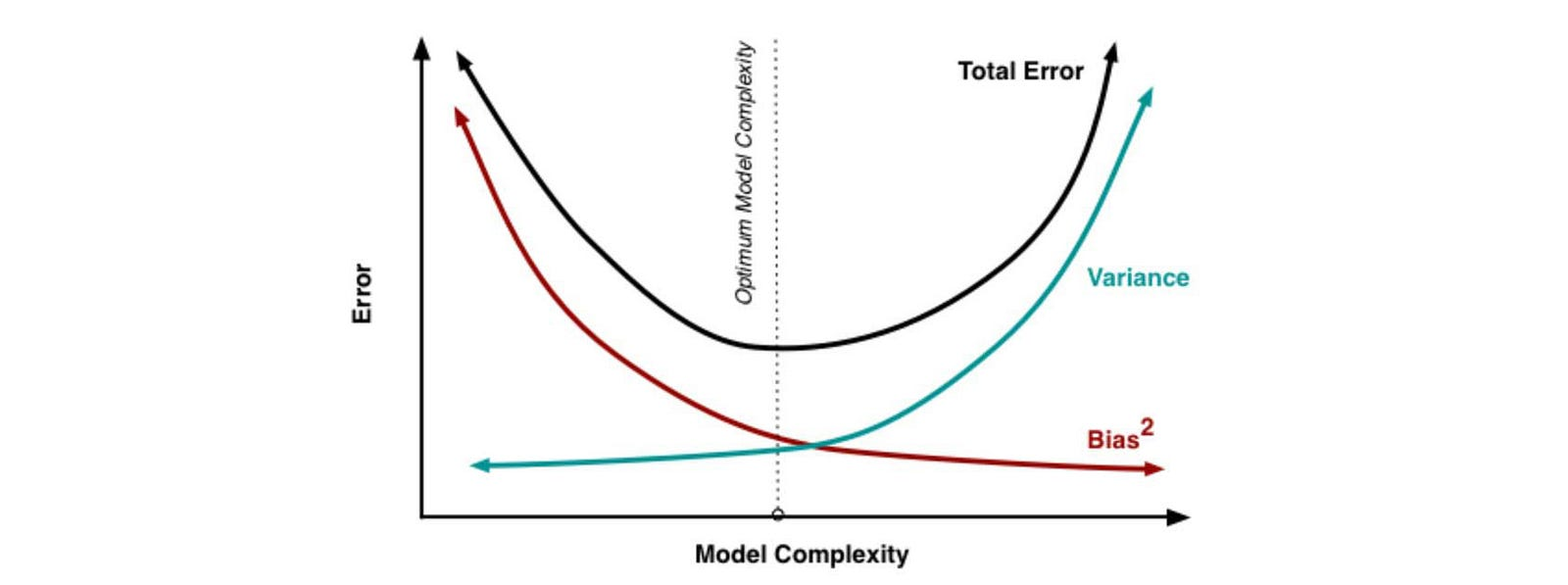Source

Let’s take a break for the fundamental concepts and interest you with some complex ML algorithms before coming back to data distribution.

### i.i.d.

In many ML problems, we assume data points are drawn from the same data distribution and the observed value does not impact the observed values of others.

This is a simple concept but the success of many ML and DL algorithms highly depends on it. Model training is more stable if data points and training batches are close to i.i.d. When we roll a dice 100 times, each data point collected is originated from the same distribution. However, if we draw from a deck of cards without replacement, the sampling distribution will change and therefore it is not i.i.d. When throwing a dice, the result is independent of previous results and therefore, throwing dice is i.i.d. Such an assumption can simplify our math in many situations. For example, the joint distribution in the maximum likelihood estimation (MLE) can be simplified as independent events.

By breaking down a model into manageable independent subcomponents, we reduce the complexity significantly. This is critical in solving such problems.

However, if we have a model trained with fair dice, the prediction will be wrong if our inference input has a different distribution, say from a biased dice. This is the covariate shift.

In some ML or DL problems, states may be highly correlated. This correlation is even higher for time sequence model. If we use gradient descent in optimizing those models, the i.i.d. assumption can hurt the performance badly which is happening in reinforcement learning.

### Expectation-maximization Algorithm (EM)

Overview

Many ML algorithms are originated from the EM algorithm. But it is one of the underrated algorithms in studying ML. To make our discussion concrete, let’s start with a clustering example. We want to find an optimal way to group 100 data points into two clusters. Here, we use the Gaussian distribution to model data distribution. The chance of a data point xᵢ belonging to a Gaussian cluster (μ, σ²) is:

Our objective is to maximize the likelihood of our observation (x₁, x₂, …) based on the Gaussian clustering models and cluster assignments.

In inference, we want to predict the cluster assignment of a new data point. In this prediction, we need θ₁ only. So how do we find the optimal θ₁ from the training data?

We start with a random guess for the clustering model θ₁ (μa, σa², μb, σb²). We infer the probability of the cluster assignments P(θ₂) for each data point accordingly. Based on

, we may conclude that for data point xᵢ=3, it has a chance of 0.2 to belong to cluster A and 0.8 chance to belong to cluster B. Then based on P(θ₂), we optimize the likelihood of the observation x w.r.t the clustering model θ₁ (arg max p(x |θ₁ )). However, θ₂ depends on θ₁ and vice versa. We are working with multiple moving parts in a circular loop.

Because of the dependency, we cannot optimize θ₁ and θ₂ simultaneously by taking both derivatives at the same time. Instead, we fix θ₁ and derive θ₂. Then we optimize θ₁ with θ₂ fixed. We repeat the iterations until θ₁ converge. In each iteration, our objective will improve. Assuming a limited precision, we are dealing with a finite space for θ₁ and θ₂. Therefore, there is only a finite step for θ₁ and θ₂ to improve and our iteration should reach a local optimal.

The EM-algorithm composes of E-step (likelihood estimation) and M-step (maximizing the likelihood). In the E-step, we fix the Gaussian models θ₁ (μa, σa², μb, σb²) of the clusters and compute the probabilities P(θ₂) (the chance xᵢ belongs to cluster A or cluster B).

Once, we compute P(θ₂), we can reconstruct the marginal likelihood P(x|θ₁) by summing P(x, θ₂|θ₁) over θ₂. Now, we have a function of likelihood w.r.t. θ₁ and we can optimize it to find θ₁.

Below is the E-step and the M-step in our example. Instead of the usual differentiation of the likelihood function, the optimization step can be done pretty simple. For example, the mean of cluster A can be computed by a weighted average of all data points with the weight equals to P(aᵢ) for the data point i. This algorithm is used in the Gaussian Mixture Model GMM. Don’t worry too much on the details as we will discuss it in here later.

Mathematical model

(Credit: the equations in this section are originated from here.)

Our GMM example is a special case for the EM algorithm. Let’s develop the math and generalize the concept a little bit more. Given xᵢ is i.i.d., data is drawn from the same distribution Ɲ and independent of each other.

Therefore, maximizing the likelihood of the observation is equivalent to maximizing the sum of log-likelihood of each observation.

Expectation-maximization (EM) algorithm is a general class of algorithm that composed of two sets of parameters θ₁, and θ₂. θ₂ are some un-observed variables, hidden latent factors or missing data. We don’t really care about θ₂ for inference usually. But if we try to solve the problem, we may find it much easier to break it into two steps and introduce θ₂ as a latent variable needed to be solved in the intermediate step. Since we don’t know both θ₁, and θ₂ initially, we need to fix one variable at a time and deriving or optimize the other variable in an alternating step. First, we derive θ₂ from the observation and θ₁. Then we optimize θ₁ with θ₂ fixed. We continue the iterations until the solution converges.

In the M-step, we maximize the likelihood of the observations w.r.t. θ₁. The log likelihood ln p(x|θ₁) can be decomposed as the following with the second term being the KL-divergence (proof).

Now, let’s make the R.H.S. as simple as possible so we can optimize it easily. First, we need to estimate q(θ₂). A natural choice will be using p(θ₂|x, θ₁) — find the probability of θ₂ given the observation and our model θ₁. By setting q(θ₂) = p(θ₂|x, θ₁), we have an extra bonus. The KL-divergence term becomes zero. Now our log-likelihood can be simplified to

Our optimal solution is the same as optimizing

In the E-step (expectation estimation step), we compute q(θ₂) and re-establish the equation for the log-likelihood ln p(x |θ₁). Optimizing the log-likelihood will be the same as optimizing the expectation of the log of the joint distribution p(x, θ₂ |θ₁), i.e. the marginal log likelihood probability ln p(x |θ₁).

In the M-step (maximization step), we find the optimal θ₁ using the R.H.S above as the objective function.

Here is the EM-algorithm:

For the solution to work, we assume we know how to model p(θ₂ | x, θ₁) and p(x, θ₂ | θ₁). The success of the EM algorithm subjects to how simple are they and how easy to optimize the later one.

As mentioned before, each iteration improves the likelihood (proof). With finite state of θ₁, our solution will converge. However, it reaches a local optimal only unless the likelihood is a convex function. In practice, even a local optimal can show us some good results.

The narrative of the EM algorithm

In some ML problems, it is intractable to solve the problem with our perceived model parameter θ₁. Instead, we can solve the problems in alternating steps by adding latent parameters θ₂. By breaking down the problem, the equations involved in θ₁ θ₂ θ₁ can be much simpler. Our objective is solving either the L.H.S. or the R.H.S. below.

If we can model p(x | θ₁, θ₂) with a simple model that can be optimized easily, we don’t need the EM algorithm. Just use MLE. But as shown below, it is not the case for GMM.

For the GMM problem, the joint distribution p(x, θ₂ | θ₁) in the EM algorithm is modeled as a Gaussian. Its log is simple. q(θ₂) is set to p(θ₂ | x, θ₁) with θ₁ fixed. It is modeled as a Gaussian also. Optimizing this formularization is easy. On the contrary, the equations for p(x | θ₁, θ₂) is very complex. Therefore, we choose the EM algorithm to solve GMM.

So if the problem can be modeled easier with its subcomponents (the joint distribution) and we know how to model p(θ₂ | x, θ₁) easily, EM algorithm will have an edge over MLE (otherwise, no).

Another example in demonstrating this idea with the PCA can be found here.

In MLE or EM, θ₁ and θ₂ may depend on each other. Therefore, we optimize θ₁ or θ₂ one at a time with the other fixed. Iterating between alternating steps, the solution will converge.

Minorize-Maximization MM algorithm

The EM algorithm can be treated as a special case for the MM algorithm. With Jensen’s inequality, we can establish a lower bound for the log-likelihood where θ₂k is one of the possible value for θ₂.

The EM algorithm becomes

Graphically, we try to establish a lower bound function 𝓛 at each iteration. We optimize the function w.r.t. θ₁ or θ₂. Then we re-establish a new lower bound.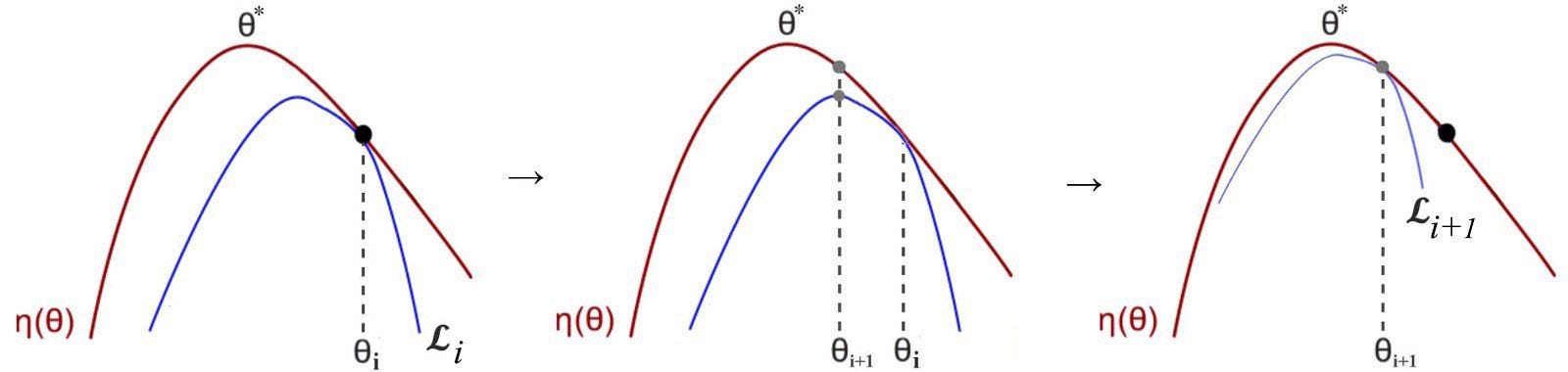Modified from source

Missing data

Previously, we introduce latent variable θ₂ to solve our optimization problem with θ₁. The EM algorithm can be used to estimate missing data in the training dataset also. For example, the training data may be collected from user surveys. But users may answer a subset of questions only and there will be different missing data in each survey. In this situation, θ₂ will be the missing data that we want to model (details).

Terms

Here is the recap of some terminology.

### Markov process/Markov chains

A first-order Markov process is a stochastic process which the future state solely depends on the current state only. When we discuss the first-order Markov process, we often reference it as the Markov process. If it is in a discrete space, it is often called the Markov chain.

The assumption of the Markov process may not be true. But even it is not true, we can model extra states to make it closer to the Markov process sometimes. In general, the Markov process is a good approximation to solve complex problems in ML or reinforcement learning.

The probability of the transition from one state to another can be packed into a transition matrix like the one below:

This transition matrix is also called the Markov matrix. Its columns add up to one — the probability of reaching a state from any possible state is one. Once, it is defined as a matrix, we can use linear algebra and eigenvector to determine its stable state if existed. Eigenvector vᵢ and eigenvalue λ of the matrix A fulfill the following relation and A can have many eigenvectors.

This stable state demonstrates the probability of being at a particular state as the time approaches ∞. The state u at time k+1 is related to the state at time k by the transition matrix A below.

If u is an eigenvector of A,

where λ is the corresponding eigenvalue.

Consider a vector v₁ in ℝ. We can decompose it using the eigenvectors of A. If v converge, v will have a stable state. The state of v at time step k+1 will be

uᵢ are chosen to be unit vectors. In order for v to converge, eigenvalues λᵢ will be smaller or equal to 1. Otherwise, ‖v‖ will continue to grow.

Say, we have an eigenvector u₁ (say 0.2, 0.5, 0.3) with eigenvalue 1. u₁ will be the stable state, i.e. we have 0.2, 0.5 and 0.3 chance to be in state 1, 2 and 3 respectively as the time approaches infinity. The solution is independent of the initial state. We end up with the same target distribution regardless of where we start. (More details can be found here.)

Random walk

Many Markov processes can be solved with random walks. Let’s say we drop off 100 shoppers randomly around the downtown area in San Franciso. We provide a transition matrix to show the probability of where the shoppers should head next. Eventually, we can spot where most interesting shops are located.

Instead of providing a global plan, the transition matrix may compose of local information only — the probability of moving ahead, turning right or turning left based on the proximity to interesting stores nearby. i.e. the transition matrix is mostly sparse. As we allow shoppers to explore, we will locate the shopping area fast. This strategy allows us to use local information to understand the general structure of the data. This strategy will work better if this local information is much easy to collect in an ML problem. We don’t need to understand the structure of the data. We don’t need to understand how the city plans its shopping districts. Just look around and see what may be more interesting.

We can build up a transition matrix and solve it analytically using eigenvector decomposition. Alternatively, we can use sampling. As we continue the iterations, our random walk will converge to the solution we want. For very large scale problem, this may be easier to execute and to compute.

Hidden Markov Model (HMM)

In many ML problems, we assume the sampled data is i.i.d. This simplifies the MLE model and makes the math much simpler to solve. But for time sequence model, states are not completely independent. If I am happy now, I will likely stay happy tomorrow.

The state of a system may not be observable or fully observable. But we can get insights about this internal state through an observable. For example, if I am happy, there is a 40% chance that I will be found at a party. But making things complicated, there is a 10% chance that I will be found at a party when I am sad. With HMM, we determine the internal state (happy or sad) by making observations — what I am doing.

HMM models a process with a Markov process which includes the initial state distribution π and the transition probabilities A from one state (xt) to another. HMM also contains the likelihood B of the observation (yt) given a hidden state. Matrix B is called the emission probabilities. It demonstrates the probability of our observation given a specific internal state.

Two major assumptions are made in HMM. The next state and the current observation solely depend on the current state only.

Given all the observable and the initial state distribution, we can compute a pretty complex equation for the probability for the internal state xt P(xt| y₁, y₂, y, … , yt). For simplicity, we will not include π in our equation. All equations assume π is a given condition, like P(y) → P(y|π).

The equation above uses the transition probability and the emission probability to compute the probability of the internal state based on all observations. Depending on the situation, three different types of questions are often asked in HMM problems.

• Likelihood: How likely is the observation based on the current model or the probability of being at a state at a specific time step.
• Decoding: Find the internal state based on the current model and observation.
• Learning. Find the HMM model.

The remaining section details the solution. Read through it according to your level of interest.

Likelihood (likelihood of the observation)

Let’s define the term α as the probability of being in a state (e.g. happy) at time t for all the observations up to t.

Here are the steps in calculating the likelihood of the observations given the model λ. We will also demonstrate these steps with an example later.

Here is an example which we start with the initial state distribution on the left. I will let you dig out the information yourself.

Decoding (find the internal states — Viterbi algorithm)

Finding the internal states that maximize the likelihood of observations is similar to the likelihood method above, except α is computed by finding the value of the maximum path. In this algorithm, we also record the maximum path (the red arrow) to track the state at time t. e.g. we are transited from a happy state H at t=1 to the happy state H at t=2.

Learning (Baum–Welch algorithm — build the model)

HMM learning is about finding the model λ given the observation. We will quote the equations from Wikipedia and adopt its notation below. Spend a few seconds in the definition below, in particular, γ & ξ. They are the latent factors in solving HMM using EM-algorithm.

γ & ξ are the latent factor θ₂ that we want to discover in the EM algorithm. γᵢ (t) is the probability of being at state i at time t given all the observation. ξᵢⱼ (t) is the probability of transiting from state i to state j at time t given all the observation.

α is the probability of seeing the observations so far (up to time t) given the state is i at time t. (forward direction). Given the state i at time t, β computes the probability of the “would-be” observations after time t. α is computed using the previous likelihood section. β can be computed similarly but instead of computing in a forward direction from t=1 to the end, we do it backward from the end t=T.Start from time=T, compute β backward using the transition prob. a and observation prob. b

With α and β (θ₁), we can compute the latent factor (θ₂) γ and ξ in the EM algorithm. The first equation below is γᵢ (t) and the second equation is ξᵢⱼ (t).

In the E-step, fixing θ₁, we compute the latent variables (θ₂) — the chance to be in state i and time t (γ) and the transition probability (ξ) from i to j at time t given all the observations. In the M-step, we optimize the model parameter θ₁ given the fixed latent variables γ and ξ. We train them in alternative steps and the model parameters will improve and converge. The following is the detail equations.

### Distribution

Let’s get back to something easier.

Expectation

For a continuous random variable, the expectation of f(x) is

For a discrete random variable

Variance and covariance

For a continuous random variable:

Variance indicates whether two variables are positively or negatively related. If it is zero, they are unrelated.

Variance can be expressed as the equation below (proof).

This formalization is useful for many proves including finding the bias and the variance for MLE estimator.

Sample variance

Sample variance is an estimator for the population’s variance by collecting m data points.

The mean of the sampling data is correlated with the data itself. (xᵢ-μ)² will be smaller than that of the population. To compensate that, we need to divide it by m-1 instead of m (proof). Note that, for the sample mean, we just divide the sum by m and it is already unbiased.

Correlation

Covariance does not account for the variance of each variable. It shows how variables are related but it does not quantify the extent well. Each variable is kind of on a different scale (or units). Correlation normalizes the covariance such that each variable corresponding to the same scale.

Gaussian distribution/Normal distribution

In a Gaussian distribution, 68% of data is within 1 σ from the μ and 95% of data is within 2 σ. The standard normal distribution has μ=0 and σ =1.

Multivariate Gaussian distribution(Gaussian Distribution with N variables)

Multivariate Gaussian distribution models multiple variables distribution using a Gaussian model. The plot below is a bivariate Gaussian distribution which two random variables are involved.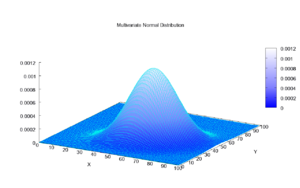Source

The Gaussian distribution for multivariate is

where Σ is the covariance matrix. Each element in this matrix records how two random variables are correlated. We can sample a multivariate Gaussian distribution by

Bernoulli distributions

The Bernoulli distribution is a discrete distribution with two possible outcomes, one with probability 𝜙 and the other 1-𝜙.

Binomial distributions

The binomial distribution is a discrete distribution from n Bernoulli trails.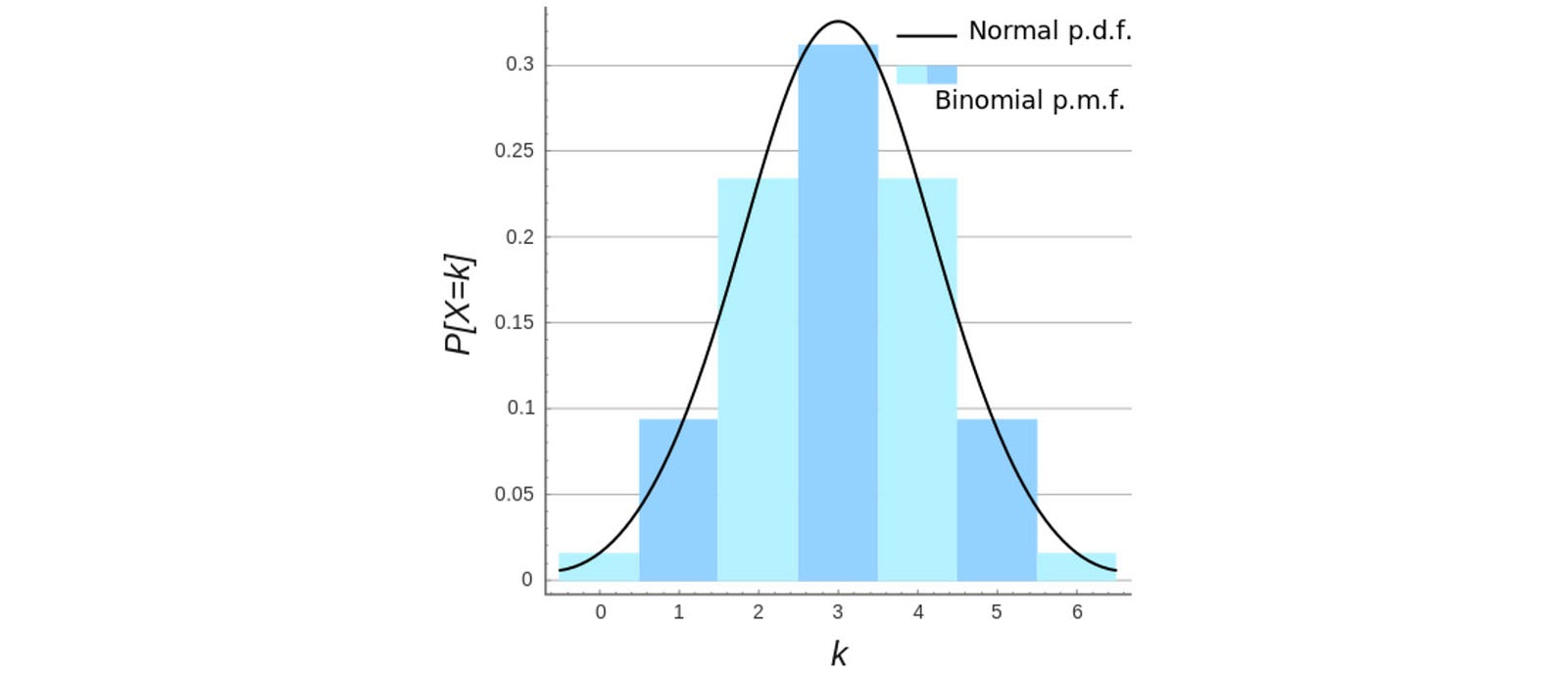Source

Variance in estimating θ in Bernoulli Distribution (proof)

Poisson Distribution

Assuming a rare event with an event rate λ, the probability of observing x events within an interval t is (proof):%

Dirac distribution

Gamma distribution

Here is the mathematical definition for the Gamma distribution.

Depend on the values of α and β, part of the equation is growing and part of the equation is decaying.

Therefore, in contrast to Gaussian distribution, Gamma distribution can generate distributions of many shapes including a single mode distribution or an exponential decay function depending on the values of α and β.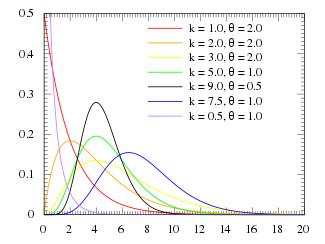Source

The expectation and the variance of the Gamma distribution are:Modified from source

If we have some estimation on the mean and the variance of the data that we want to model, we can reverse engineering the equations above to find α and β.

Unlike Gaussian distribution, by definition, the PDF is zero for x is negative. This can be a nice constraint in modeling some real problems.

Beta distribution

The beta distribution is defined as

Here are different shapes of data distribution using different parameters of a and b. As shown below, Beta distribution can model U shape and uniform distribution.

The mean and the variance of the Beta distribution are: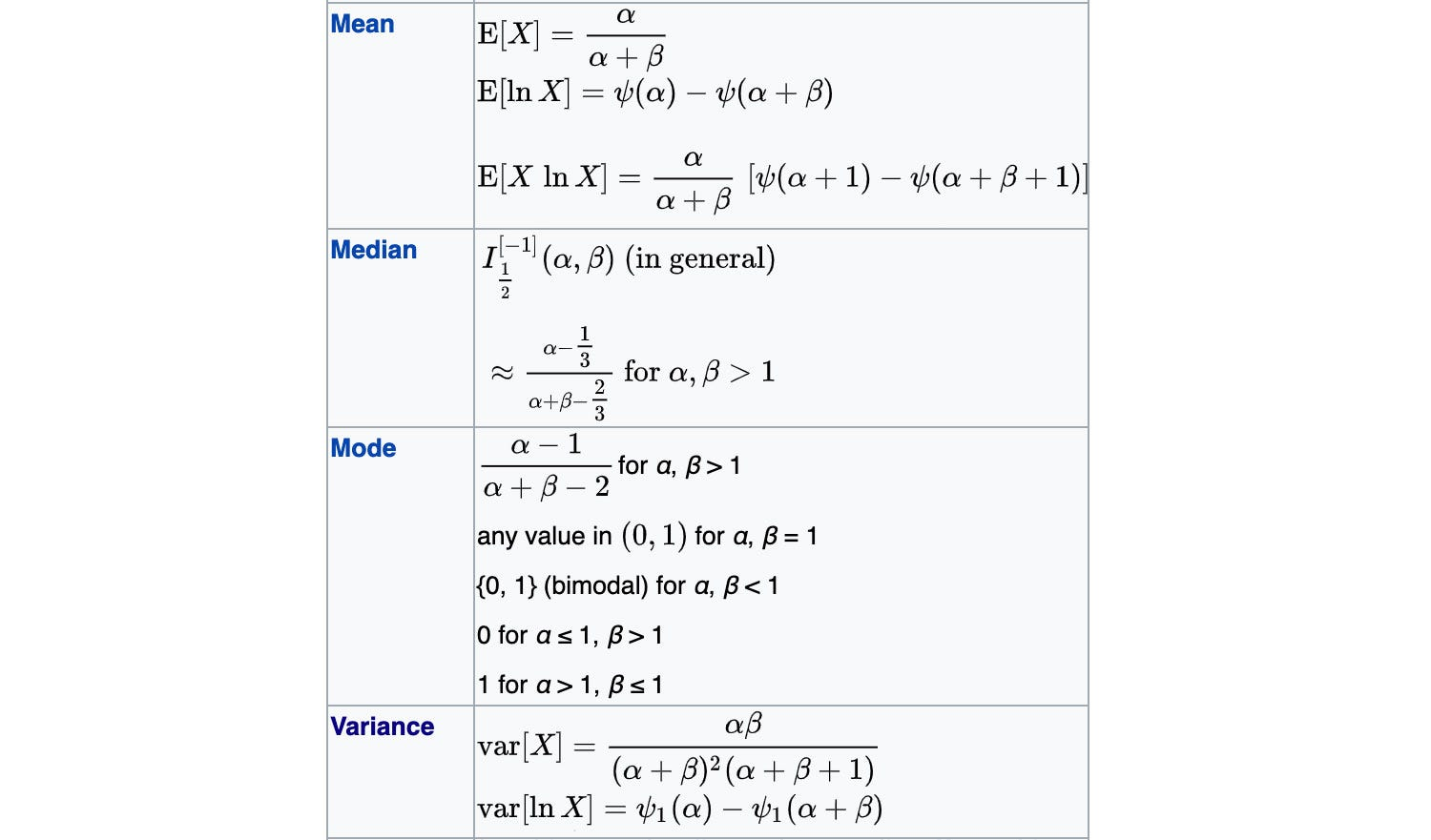Modified form source

We will demonstrate the application of beta distribution in Bayesian inference later.

Chi-squared distribution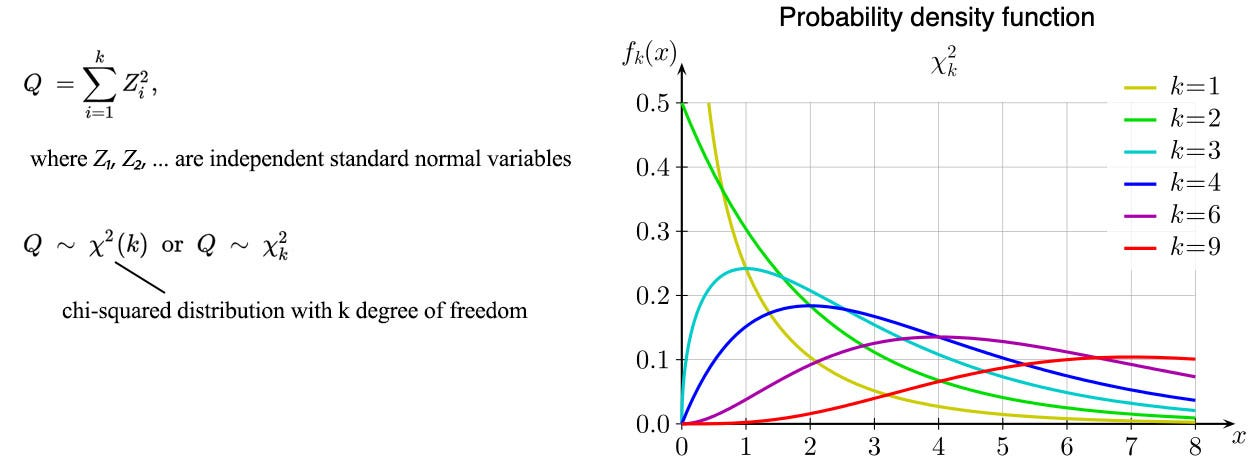Modified from source

Exponential and Laplace distribution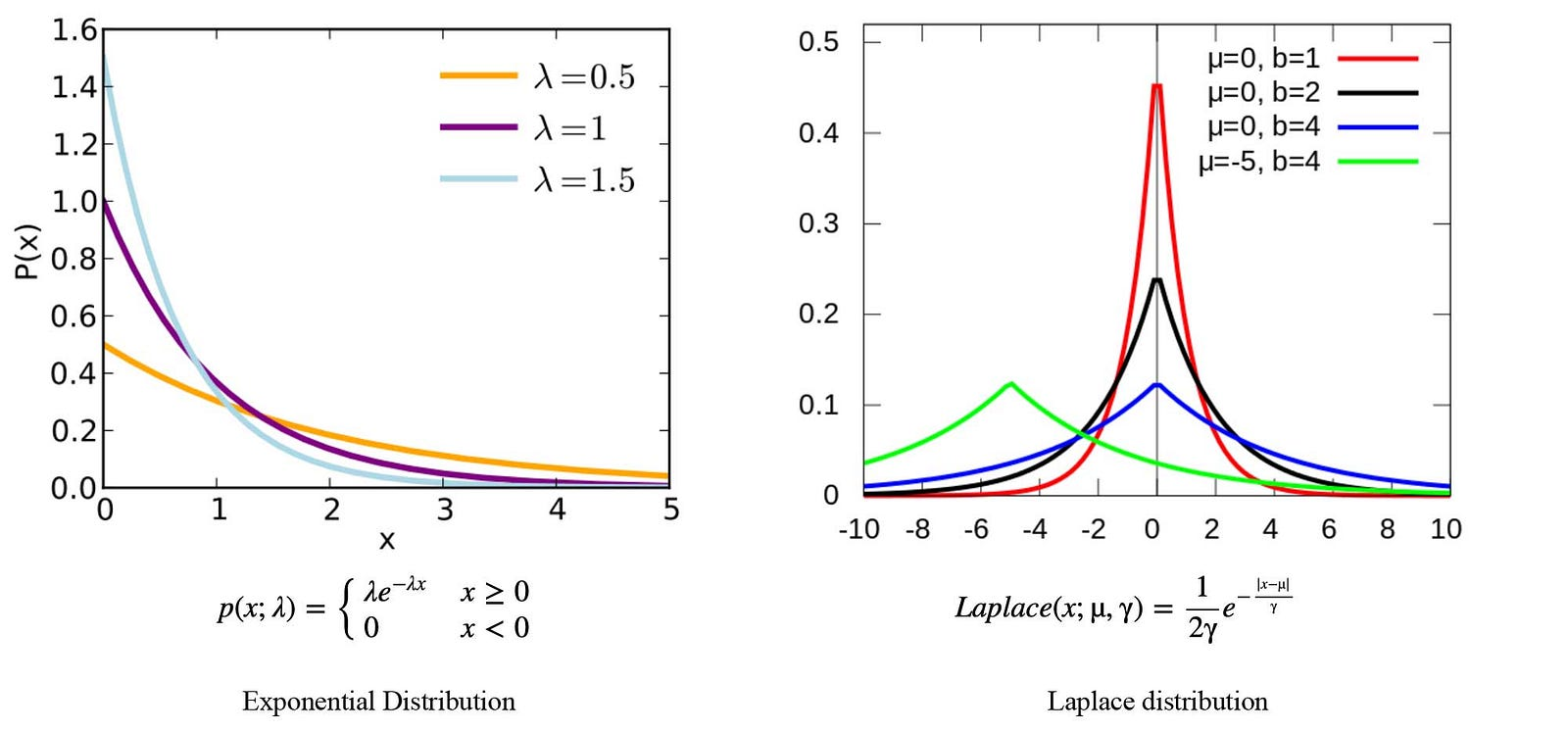Modified from Wikipedia

Recap

Central limit theorem

Consider rolling dice once, the value of the throw is uniformly distributed if the dice is fair. Let’s throw the dice many times and each time we average the result. We repeat the experiment many times and collect all the aggregated results. When we plot these results, we will realize the results are Gaussian distributed. The central limit theorem says that we can have many independent random variables (say each represents the result of a throw). When we add them together and normalize it (average it), the normalized results after many trials tend to be Gaussian Distributed. The theorem extends to any distribution for the independent variables. So no matter whether the dice is fair, biased or with any data distribution, the normalized results are Gaussian Distributed. This implies that individual variables may have different data distribution, we can use a Gaussian distribution to model its aggregate results.

If the random variable is already Gaussian Distributed with variance σ², the normalized results will be

i.e. the variance of the aggregated result drops as it is average from a larger sample size.

### Bayesian inference

Frequentist inference draws conclusions from the frequency of events. If we get two heads from flipping a coin twice, p(head) equals 100%. However, a frequentist will unlikely publish such a result because the sample size is too small. The result may be just by chance and it is too early to spot any trend.

Bayesian inference uses Bayes’ theorem to derive a posterior distribution from the likelihood and a prior belief.

It combines our belief with the observation to form a posterior that becomes our new belief. For example, from the previous measurements (or observations), the dynamic model on how a car position changes and even naive believe, we form a prior belief on the current position of a car. Given the current sensor reading (like GPS), we form a likelihood of our sensor reading given different locations. Using Bayes inference, we can derive the probability distribution P(H|E) of the car location given the sensor readings.

We can turn the posterior into the prior for our next iteration when new observations are made. If the sample size is small, the likelihood curve is wide with a lower peak. We have not drawn enough data to rule out many possibilities. Therefore, the posterior will resemble the prior belief if it is strong (narrow and peaked). When more data is collected, the likelihood will get pointy and the posterior distribution will get closer to the likelihood curve.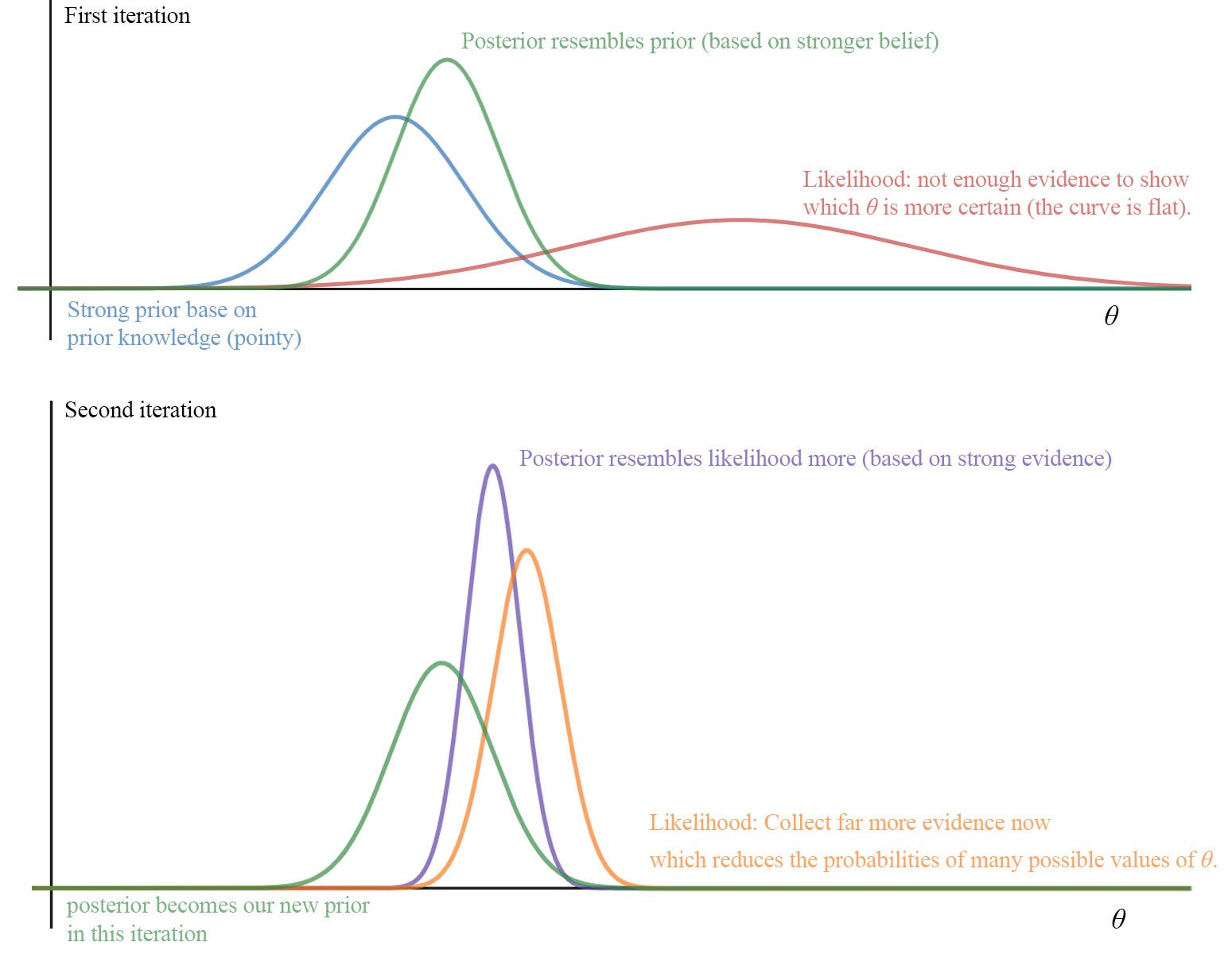illustration purpose only

Frequentist v.s. Bayesian

Frequentist applies the maximum likelihood estimation to find an optimal model parameter in explaining the observations. The Bayesian put the spotlights on the model parameters and calculate the posterior using the Bayes’ Theorem. It calculates the probabilities of all different models given the observation. Of course, this can be extremely complex for high dimension or large continuous space. Therefore further modeling on the likelihood and the prior are applied, or we can solve the problem by sampling or approximation.

Depending on the way how samples are collected, it may be easier to answer P(x|y) than P(y|x). Sometimes, the probabilities are easy to model in the opposite direction. For example, P(y | x, θ) and P(θ) are often modeled with Gaussian or Beta distribution. Below is an example of the Bayesian linear regression. We ignore the denominator P(y |X) in the Bayes’ Theorem because it is not a function of θ and can be dropped when we optimize the posterior w.r.t. θ. In practice, P(y |X) or P(X) is often very hard to compute, so this is a very nice simplification in optimizing the posterior.Linear regression
If you cannot solve P(y|x) easily, transform the problem with Bayes’ Theorem and try your luck in the opposite direction P(x|y).

In the Bayes’ Theorem, we have relatively high freedom in choosing the model for P(θ). But not every choice is equal. Since the posterior can be used as the prior in our next iteration, we may want the posterior and the prior to be in the same family of distribution. So we can apply the same math for the next iteration and we can compute the solution analytically. A prior is a conjugate prior if the corresponding posterior belongs to the same class of distribution. For example, both are Gaussian distributed.

Let’s look at them in detail but we will model the prior with a Beta distribution.

Bayesian inference with Beta distribution

Multiplying two probability distributions (the prior and likelihood curve above) is not easy in particular when both are continuous. But if we can model both curves mathematically, like using Gaussian distribution or beta distribution, we can solve them analytically. For example, Binomial distribution can be model by a beta distribution. The conjugate prior that we want to use for binomial or Bernoulli likelihood will be the beta distribution. The posterior will be beta distributed and easy to compute.

The detailed example demonstrating the Bayesian inference with Beta distribution can be found here. Here is the skeleton on finding the posterior using beta distributions. We use beta distributions for both P(data|θ) and P(θ). The posterior P(θ |data) will be beta distributed also.

Let’s consider the infection rate for a person exposed to a virus. If we have no prior knowledge, we can start the prior with a uniform distribution (left below). The posterior in the Bayesian inference will resemble the frequentist result since our belief is weak.

Otherwise, we can start with some prior knowledge based on past experience, knowledge or even gut feeling. However, if our belief is off, we need to collect more data to gradually reshape the posterior to the correct curve.

While we can start with a non-informative prior, the strength of Bayes inference depends on how easy and reliable to form our prior belief.

Gamma distribution as a conjugate prior

We can use a Gamma distribution as the conjugate prior if our likelihood can be modeled by a Gaussian distribution.

The Gaussian distribution for our likelihood p(x|θ) can be expressed in the following form.

Apply the Bayes’ Theorem, we can derive the posterior in the form of Gamma distribution also.

Prediction & regularization

With Bayes’ Theorem, we calculate the posterior for the model θ given the observations. Prior is our prior knowledge. If we assume θ to have a zero-centered Gaussian distribution. This prior p(θ) turns into an L2-regularization term in the objective function (details). Conceptually, p(θ) can be viewed as a regularization factor in penalizing a cost function if θ deviates from our prior belief. As shown below, it can be a very complex function.

To make a new prediction, we use the posterior p(θ | X, y) in our training as p(θ). Then we find the marginal probability p(y|x₀) by integrating over θ. This is Marginal inference. We compute the probability of a variable by summing everything else out.

### Graphical Model

In a Graphical Model, we apply domain knowledge in modeling data dependency. Probabilistic graphical modeling combines both probability and graph theory in solving ML problems.

Bayesian network

A Bayesian network represents variables and their conditional dependencies using a directed acyclic graph (DAG). Variables can be positively dependent, negatively dependent or independent. For example, if it rains, we have a smaller chance to turn on the automatic sprinkler.

In our model, rain impacts whether the automatic sprinkler should be on or off. The grass is wet if it rains or the sprinkler is on. However, in our model, the wet grass has no impact on the chance of rain or the chance of turning the automatic sprinkler on. Therefore, we can further simplify the chain rule below for the joint probability.

For example, P(S|R, W) becomes P(S|R) according to our Bayesian network model. The conditional probability below is calculated by Bayes’ Theorem and marginal probability.Modified from source

And the joint probability on the R.H.S. is calculated with conditional probability.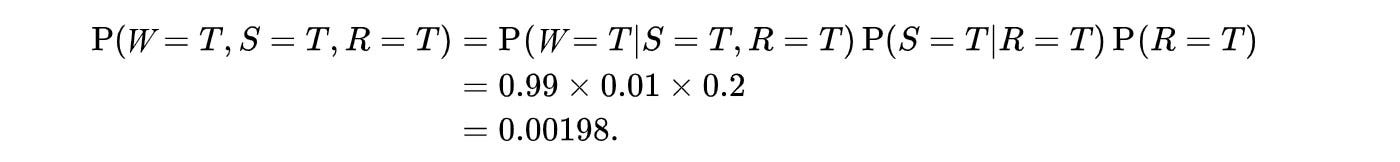Modified from source

As a reference, the data needed in the equation is collected as follows: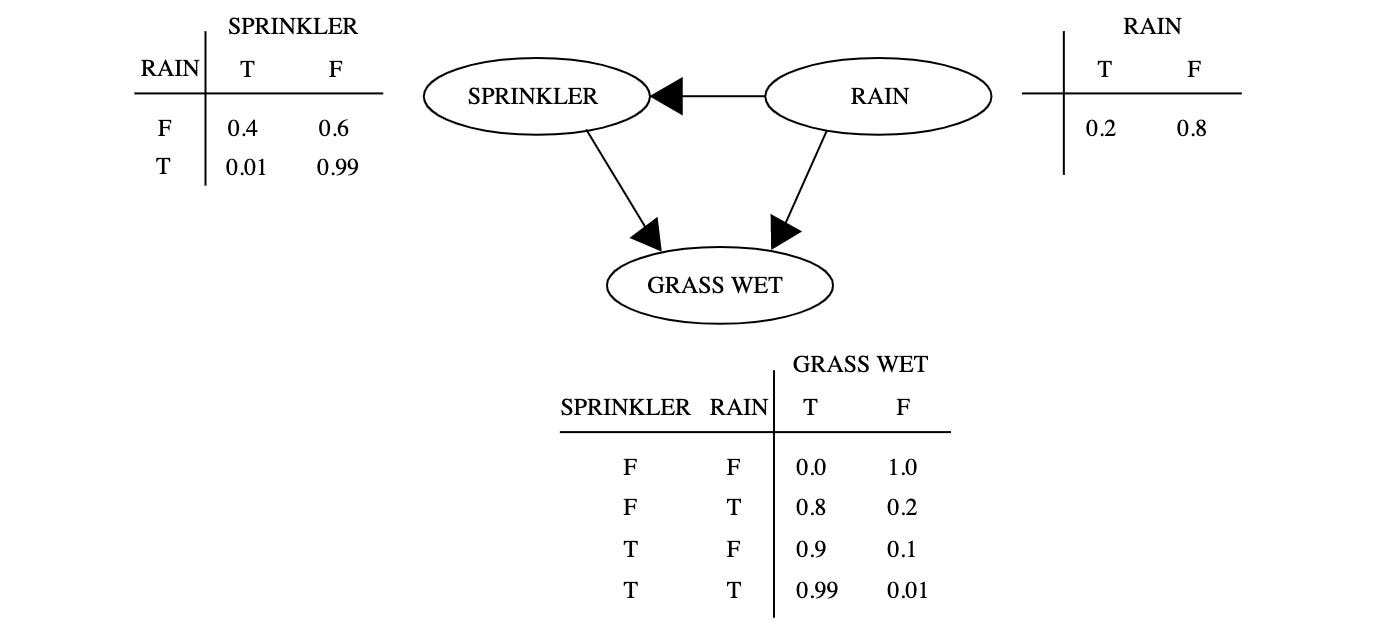Source

### Sampling-based inference

Probabilistic models are usually complex. If we cannot solve them analytically or efficiently, we can approximate the solution. One popular approximation approach is through sampling.

Monte Carlo Method

In Monte Carlo, where gamblers gather, probability, statistic, and psychology govern. A Monte Carlo method is a fancy name for analyzing a stochastic process through repeated sampling.

Monte Carlo Integration

For example, in marginal inference or MAP, we need to compute the marginal probability

Or we need to compute the expectation of f(x) with x having a distribution p.

This can be estimated by drawing N i.i.d. samples for p.

The estimated expectation is unbiased with variance inverse proportional to N.

Markov Chain Monte Carlo

As mentioned before, knowing the posterior equation is not good enough to solve many problems, for example, the expectation. If we cannot solve it analytically, we can sample points and calculate the specific values for f(xᵢ). If we collect enough data, we can use the results to estimate the expectation. Similarly, we can use this concept to estimate an integral present in many equations or finding an optimal path for maximum rewards. Solving it analytically is often too hard otherwise.

In the example above, we sample θ uniformly alone the x-axis. For each θ value, we find the corresponding prior and likelihood value and multiply them together to find the corresponding posterior value. For simplicity, we ignore the marginal probability here but can be estimated through sampling or ignored in some cases. But the complexity remains too high for high-dimensional space. For Google AlphaGO to beat the GO champions, the secret recipe is how to sample data in high dimensional space smarter.

In Markov Chain Monte Carlo (MCMC), we adopt the Markov chain mechanism and use the transition probability to sample the next state. Optionally, we can perform an acceptance test. If the test fails, we rollback the state. In summary, MCMC is a general concept in using Markov Chain to select samples and use sampling to compute results or actions for a stochastic process. MCMC does not specify the exact probability distribution or the optional acceptance test. It is left for specific methods to determine.

Metropolis-Hastings algorithm

Metropolis-Hastings algorithm is one of the most popular MCMC methods. First, let’s pick a proposal distribution g which we use to determine which state to explore next. One possibility is the normal distribution. i.e. we pick a neighbor with chances decreased exponentially as it moves away from the current state. (Other choices can be used in this algorithm.)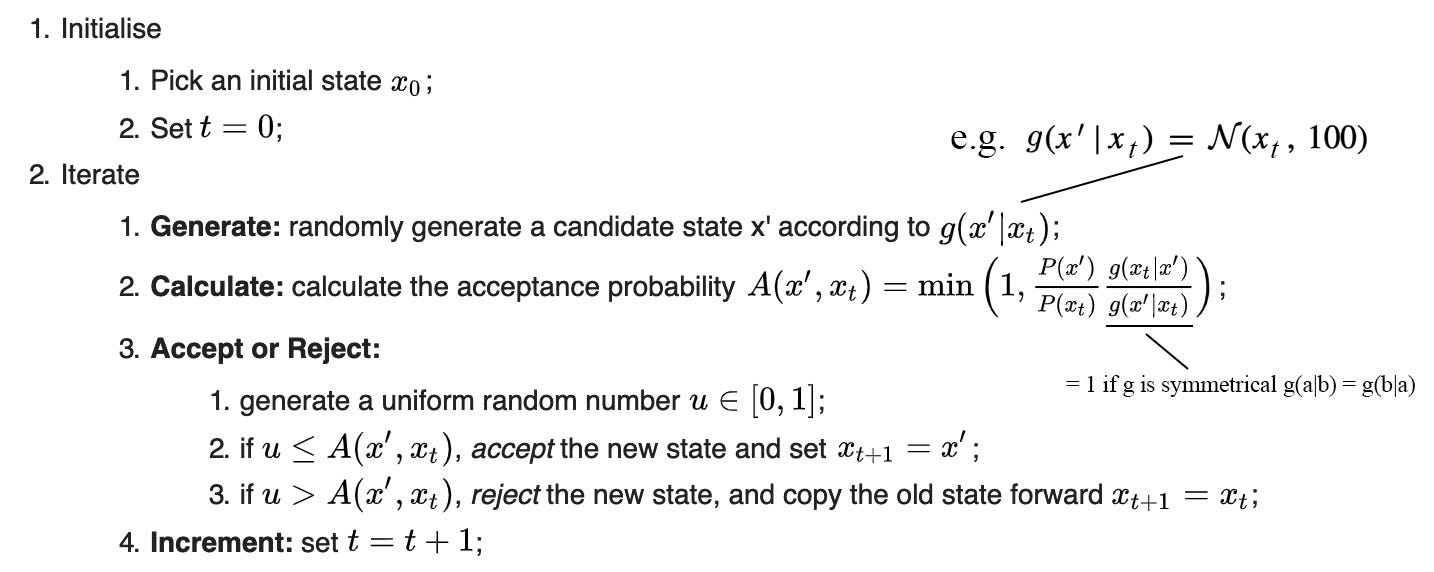Modified from source

Next, we establish an acceptance probability A in determining whether we will select the candidate state for sampling or select another neighbor again. Basically, if the candidate state has a higher probability than the current state described by the target distribution p, we give it a higher chance to be sampled. As shown below, the solid line is the target distribution p. As the number of iteration increases, the collected sample points resemble the target distribution using the Metropolis-Hastings algorithm. So we can use the collected sample to calculate the expectation or to reconstruct the distribution.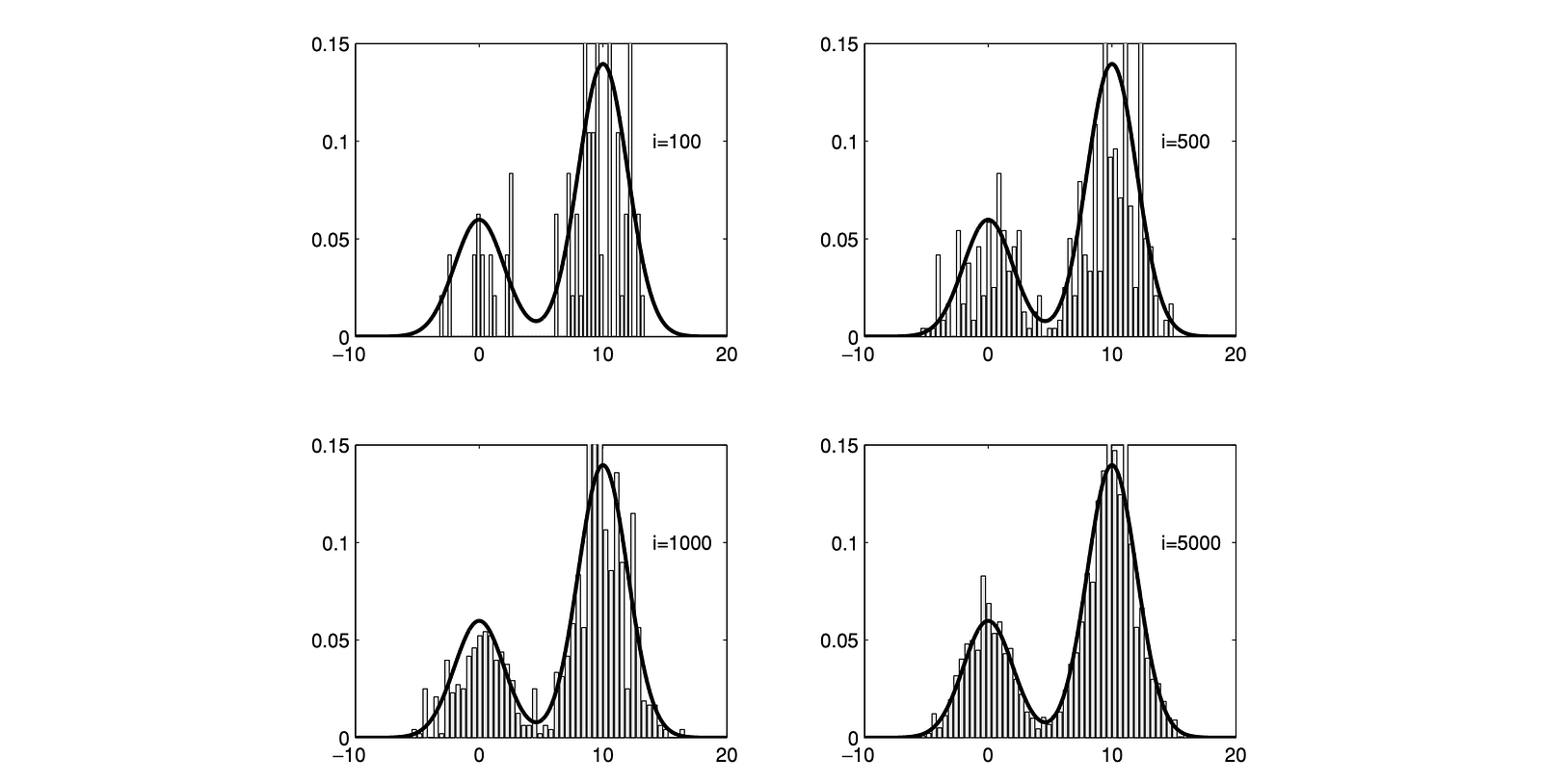Source

In the Metropolis algorithm, we pick the proposal distribution g to be symmetrical. i.e. g(a|b) = g(b|a)). The acceptance probability A will be simplified to

Gibbs sampling

Gibbs sampling is another MCMC method. Let assume each data point is a D-component vector. We sample one component at a time with other components fixed.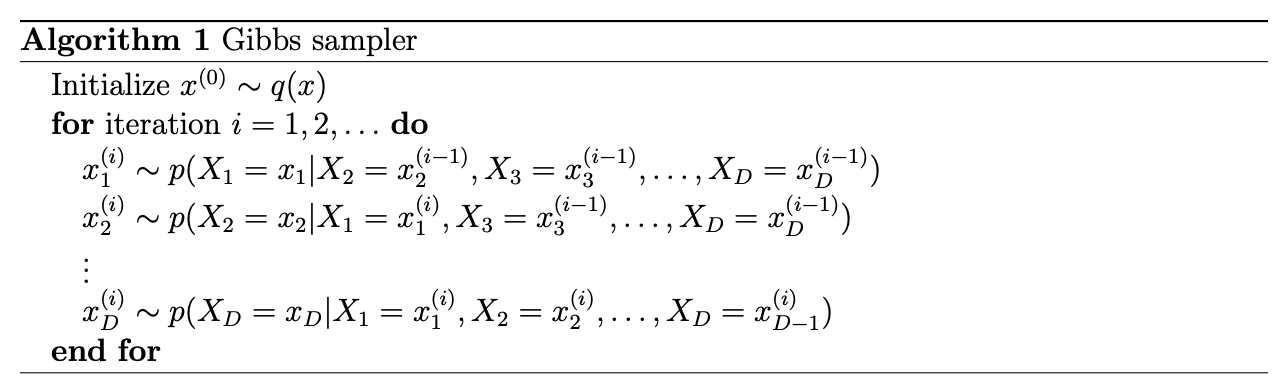Source

On the left diagram below, we plot the sampled points for the first 50 iterations. This example is in 2-dimension space (D=2). In each iteration, we change one dimension only. Therefore, it moves vertically and horizontally in each alternating steps. We sample 1000 points from the original data distribution p and the distribution for the 1000 sampled data from the Gibbs sampling. Both plots look similar.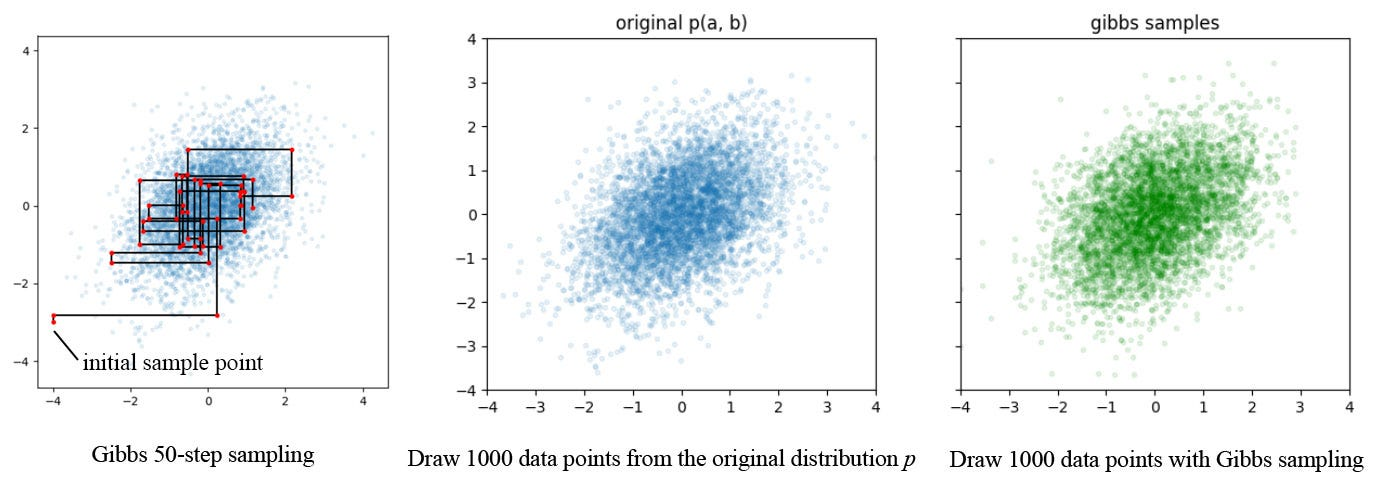Program output with code originated from this source

Importance sampling

We can compute the expectation 𝔼p[f(x)], with target distribution p for x, using a proposal distribution q.

i.e. we sample f(X) with the distribution q to calculate the expectation of f with distribution p.

So what is the motivation? Let’s take an example in the Seaquest game. Say we already sample the total game score based on actions modeled by the proposal distribution q (chance of moving the submarine up/down/right/left). What is the expected total score if we take a new action plan with distribution p?

In practice, as we change any game plan, the expected total score for each action changes. We need to resample all the possible future moves many times to compute the expected total score. But if we keep p ≈ q, we can reuse the information from q to estimate p using importance sampling. In this case, we can perform the complete resampling less often (say only 1 out of 4 times).

For the estimation to have the minimum variance, the sampling distribution q should be:

Intuitively, if we want to reduce the variance of our estimation, we want to sample data points more frequently in areas where |f(x)| is higher.

### Variational inference

Even the posterior is known, sometimes, it is hard to be manipulated or use it for the prior in the next iteration. Sampling is one method to approximate a solution and variation inference is another popular method to approximate a distribution. For example, we can approximate the posterior below with a Gaussian that is much easier to analyze.

Therefore, we want to find q that belongs to a specific class of distribution (say the Gaussian) that minimizes the KL-divergence below.

Let’s look at the typical problem in applying the Bayes’ Theorem. The marginal probability p(X) is hard to compute.

So, if we don’t need to renormalize the distribution by Z, it would be nice.

As shown above, Z is a constant w.r.t. q. Therefore, we don’t need to renormalize the distribution in finding the optimal q.

Evidence lower bound (ELBO)

Let’s define J as

The lower bound for log Z(θ) is -J which is called the evidence lower bound. It establishes a lower bound of the log-likelihood of the evidence X. From the equation above, minimizing the KL-divergence is the same as maximizing the evidence lower bound (ELBO). ELBO is often written as:

### Derivative

Jacobian matrix & Hessian matrix

These matrices are the first order and the second order derivatives of f respectively.

This notation is called the numerator layout. Hessian matrix is symmetrical. The upper bound for a quadratic equation with the Hessian matrix and a vector v is

Below, we use a different notation called denominator-layout notation. It is the transpose of the numerator layout.

Here is the result in differentiating a vector and a matrix in such notation.

### Matrix Decomposition

Graphical interpretation of the component analysis

We can represent a 2-D vector x by projecting x onto the x-axis and y-axis. So a data point can be represented as (xᵢ, yᵢ). The choice of x-axis and y-axis is pretty arbitrary. Instead, we can select a unit vector q and calculate the projection of x on q. The projection vector will be qqᵀx with its magnitude equals qᵀx.

In ML, we extract features from a high-dimension space into a low-dimension latent space (say with k-dimension). Conceptually, we project x onto k different vectors qⱼ. The choices of qⱼ are important. If it is done correctly, we can use fewer components in representing information. For example, if we choose q₁ and q₂ below, we may ignore the q₂ component (the blue dots). They may be too small and we can ignore them. That is not true if we pick the x-axis and y-axis.

SVD decomposes a matrix into independent components. All q selected in SVD are independent of each other (orthogonal), i.e. the extracted features are not correlated. Conceptually, SVD picks the first q that minimizes the least square error below if the rest of the components are dropped.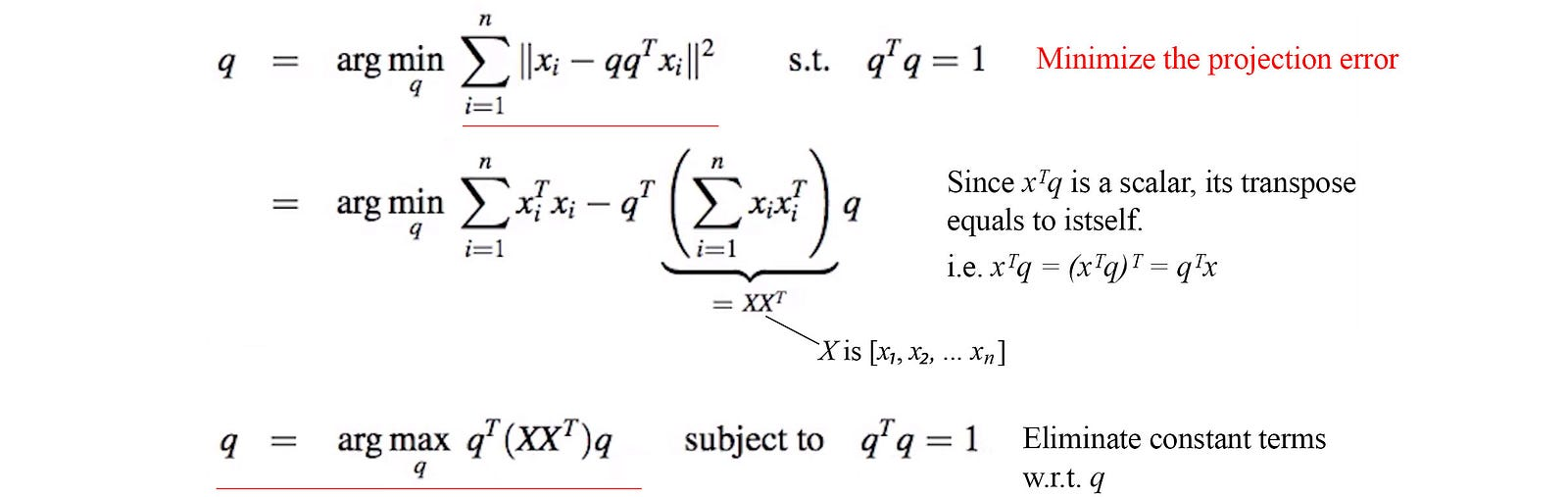Modified from source

XXᵀ is symmetrical. The optimal q (named q₁) will be the eigenvector for XXᵀ with the largest eigenvalue λ or the largest singular value σ (λ = σ²). (proof).

Then we can pick the next component based on the same principle with the condition that q are orthogonal to each other. The selected q₂ will have the second largest eigenvalue. We can continue the process until we run out of eigenvectors. But the description here is just for demonstration. We don’t solve the problem sequentially. It just helps us to understand the principal of the feature extraction behind SVD.

Singular Value Decomposition (SVD)

SVD is presented differently in linear algebra. It is the same method but from a different angle. Any matrix A can be decomposed as (proof)

where U compose of uᵢ — the eigenvectors for AAᵀ and uᵢ are orthogonal (perpendicular/independent) to each other. (uᵢ is equivalent to qᵢ in the last section.) Similarly, V is composed of eigenvector vᵢ of AᵀA which is also orthogonal to each other.

From the equation above, A can also be written as

uᵢ and vᵢ are unit vectors. So when we evaluate the importance of the decomposed components, we can ignore those terms with very small σᵢ.

If we keep only the topmost k terms with the largest σᵢ, we effectively reduce the rank of A into k, i.e. the extracted features is in k dimension only. We effectively reduce the dimension of the input with the consideration of the importance of each principal component. That is what PCA does.

Principal Component Analysis PCA

As shown below, PCA simply truncates those insignificant terms in SVD (terms with very small σᵢ).

Intuitively, two input features may be so correlated that you can create a new feature to represent both. For PCA, we want to find k independent features to represent our data.

PCA Example

In ML, SVD decomposes a matrix containing training data into independent features. For example, the row of the matrix contains the user ratings on a movie. The column contains the movie ratings from a user.

If we pick the top K eigenvalues of AAᵀ, its corresponding eigenvectors is equivalent to the top K optimized qk vectors below: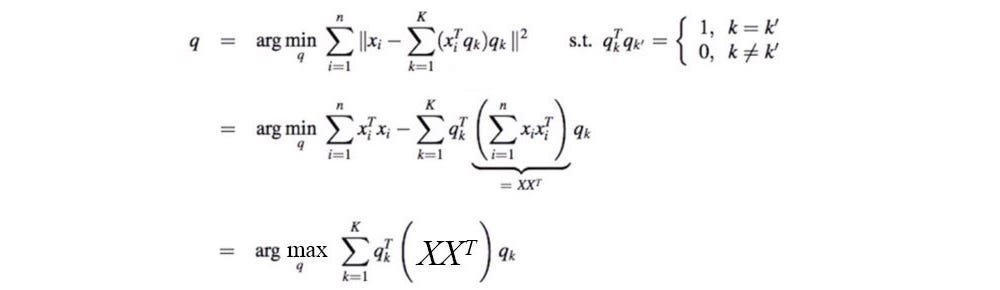Modified from source

In other words, to find the optimal q, we find the top eigenvalues of AAᵀ. The corresponding eigenvectors are the most important principal components we care about our data. If we multiply the input with these principal components, we can reconstruct the original data the best with the least number of components.

We can concatenate qᵢ to form the matrix Q. We can derive the latent features of user by multiplying Qᵀ with a user’s movie ratings. (qᵢ is N × 1 where N is the number of movies, and Q is N × k.)

SVD discovers the patterns (principal components) on user ratings. We can imagine some of the principal components may represent the genre of the movie or the years of the release. For example, the first component in zᵢ may indicate whether a user likes comedy.

Probabilistic PCA

In SVD, we decompose X to USVᵀ. Probabilistic PCA models X≈WZ. We will use the EM algorithm to learn W and Z which Z can be treated as the latent feature of X. Unlike SVD, W does not need to be orthonormal. Columns do not need to be of unit length or perpendicular to each other.

First, let’s assume the latent variable zᵢ is a zero-centered Gaussian distribution. With W, we can reconstruct the original data X by WZ where x is modeled by a Gaussian also.

Z is the latent variable θ₂ in the EM algorithm and W is θ₁. Our objective is

In the E-step, we compute the Gaussian distribution for q(zᵢ).

In the M-step, we optimize

Without proof here, the algorithm is: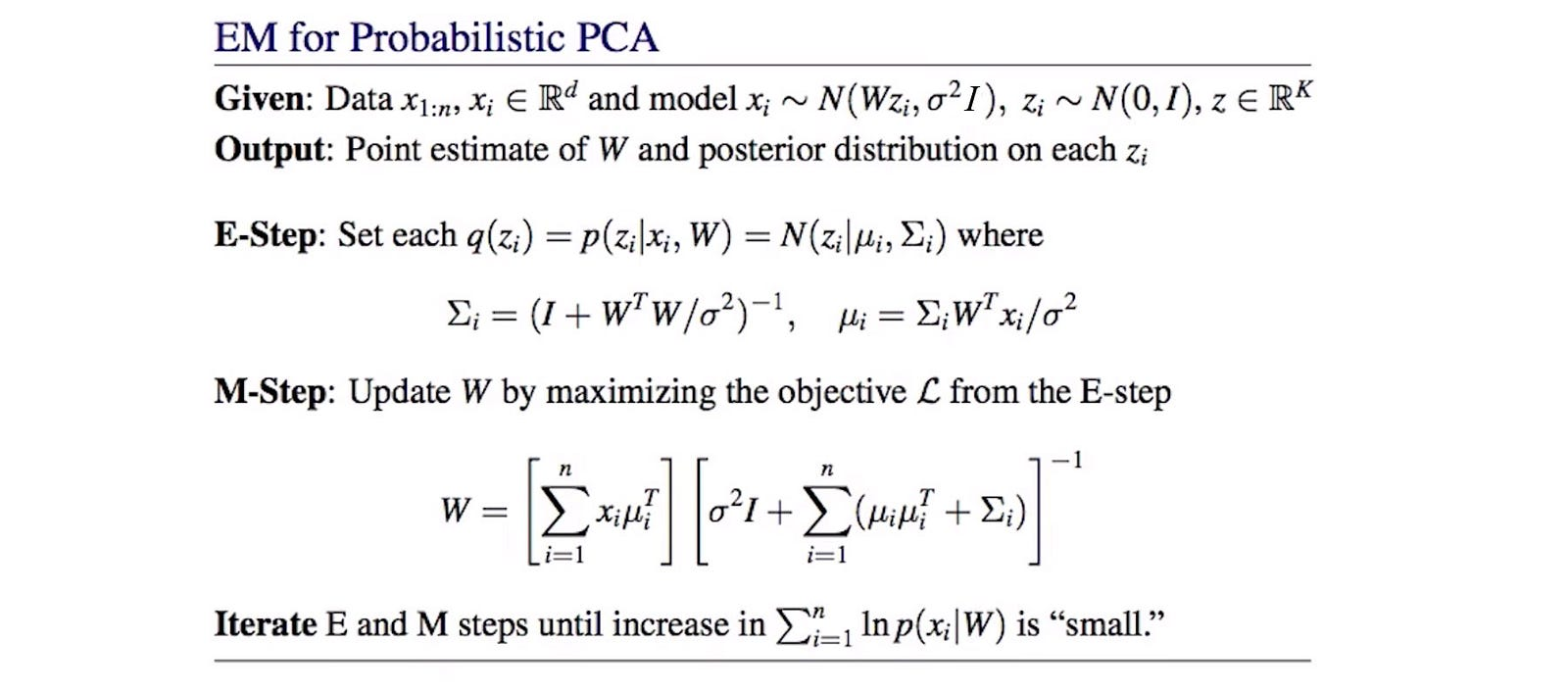Source

Kernel PCA

From one perspective, PCA finds a set of vector q that maximize qᵀXXᵀq. Since XXᵀ is symmetrical, q will be the eigenvectors of XXᵀ with the largest eigenvalues (details).

So the problem statement can be rewritten as finding the eigenvectors with the largest eigenvalues.

In the article on ML algorithms, we replace XXᵀ with a kernel to map the input to a higher dimension. This allows us to create a linear boundary to classify data that is not linearly separable in the low dimension space (details). On the contrary, PCA is often considered as a dimension reduction technique. So both technique seems to go in the opposite direction. However, sometimes, we need to go big before go small. Going to a high-dimensional space allows us to cluster information with a simpler and clear boundary. Once the information is clearly cluster, it will be easier to map it to a lower dimensional space. This is the motivation behind PCA kernel. Let’s start with the following equation.

After some manipulating, we get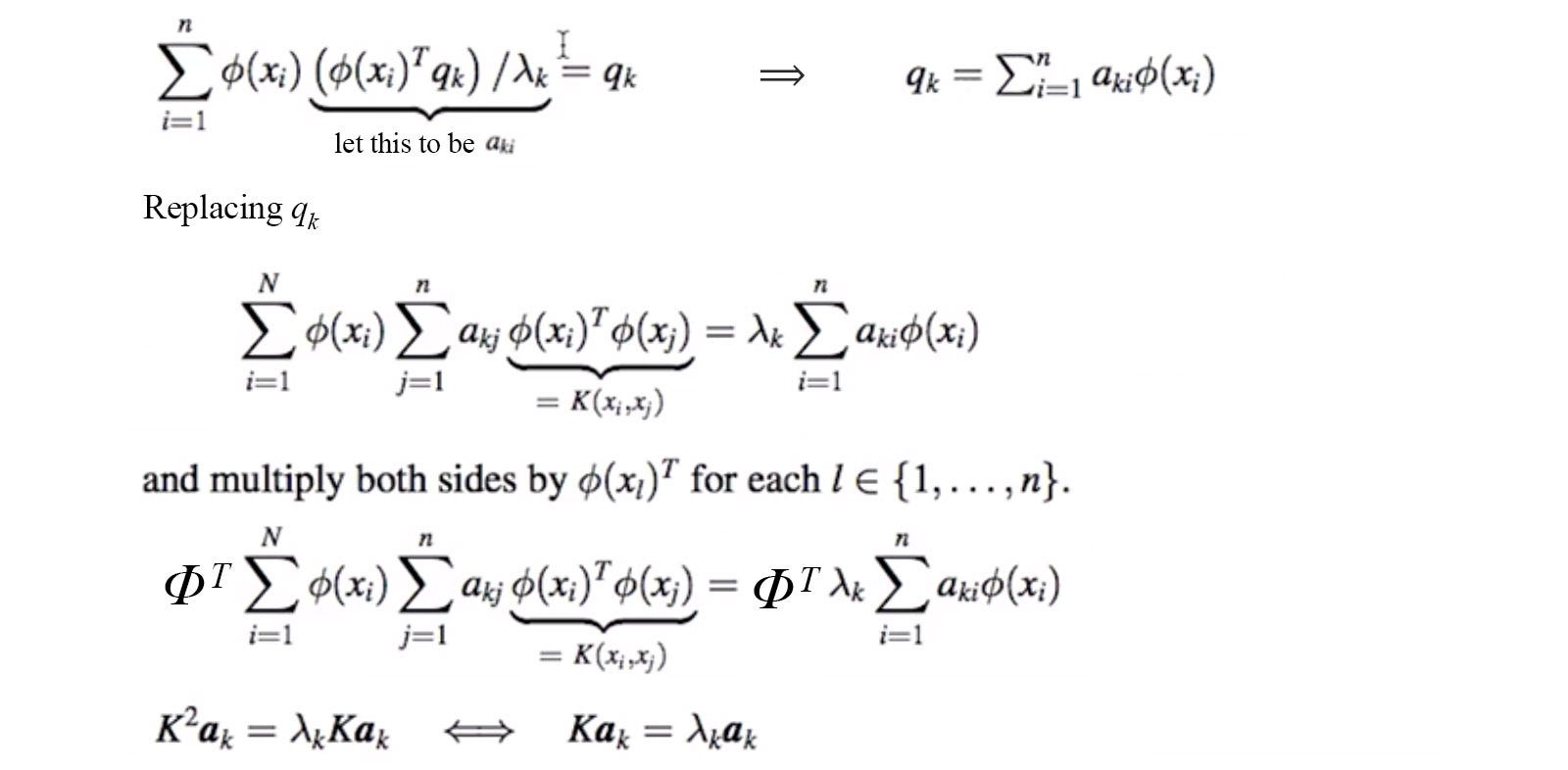Modified from source

So given the matrix K holding the kernel result, we can find aᵢ by finding the eigenvectors of K. Next, we will define the kernel function as a Gaussian distribution. The corresponding latent factor for x can be computed as: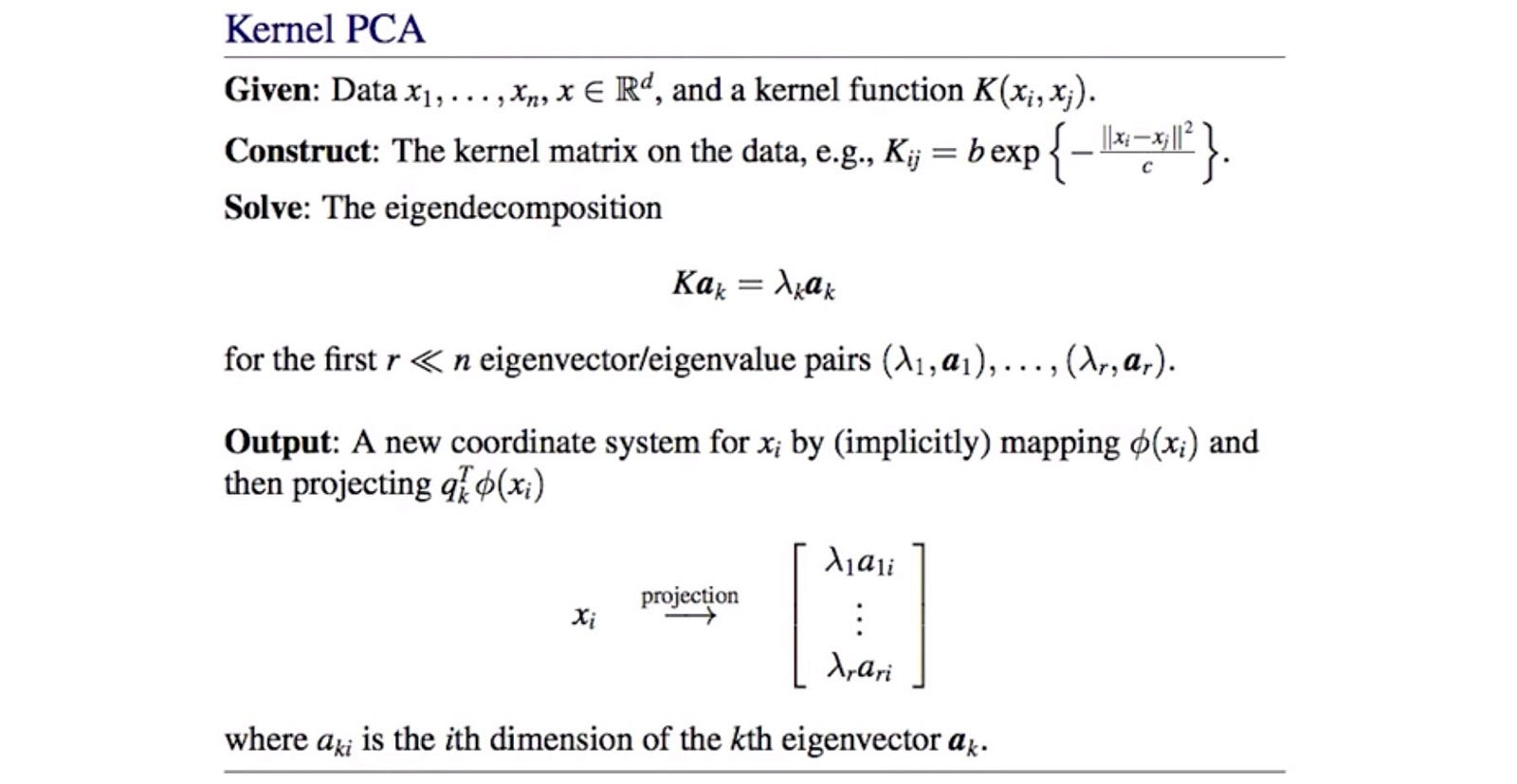Source

To make a prediction for a new input x₀ using Kernel PCA: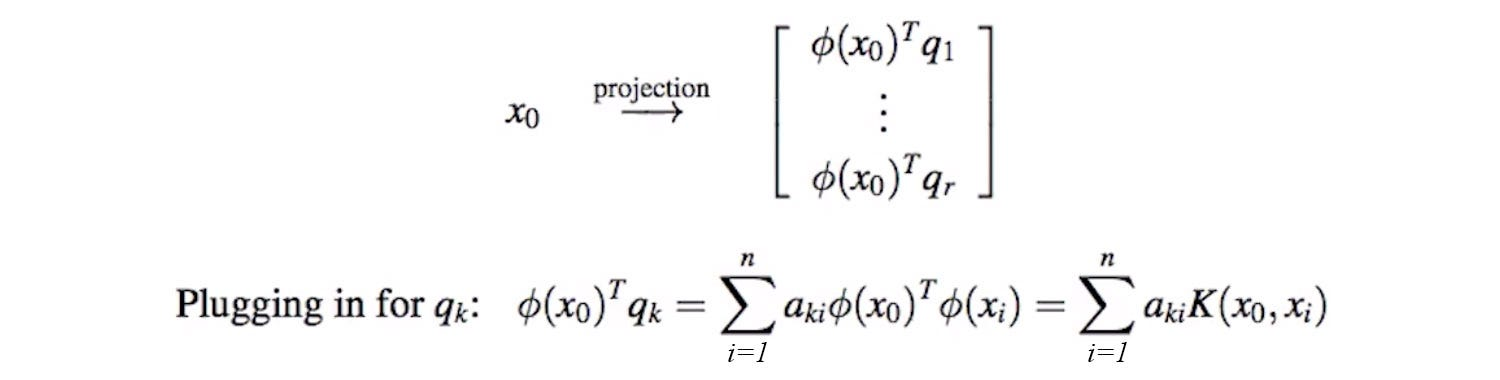Source

Moore-Penrose Pseudoinverse

For a linear equation system, we can compute the inverse of a square matrix A to solve x.

But not all matrices are invertible. In ML, it will be unlikely to find an exact solution with the presence of noise in data. But the solution for x can be estimated as,

where

Cholesky decomposition

The Cholesky decomposition of a Hermitian positive-definite matrix A is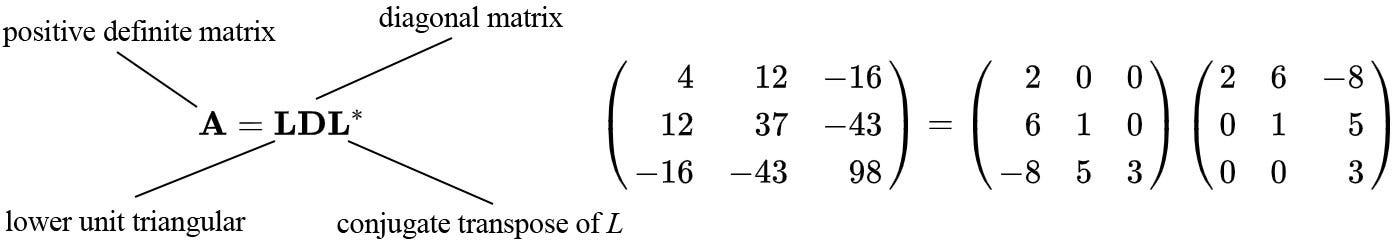Source

A Hermitian matrix is a square matrix that equals its transpose conjugate. A transpose conjugate takes the complex conjugate of each element and then transpose the matrix.

A covariance matrix is symmetric (a special case for Hermitian if values are all real) and semi-positive definite. Therefore, Cholesky decomposition is commonly used in ML for easier and more stable manipulation.

### Statistical Significance

Null hypothesis H₀ is a statement that there is no relationship between two measured phenomena, for example, no correlation between wealth and happiness. This idea can be further extended for statements that we want to nullify and repute. A null hypothesis will be rejected if the observed data is statistically significant, i.e. it is very rare to observe the collected data if the null hypothesis is true. For example, if we see 100 heads in flipping a coin 100 times, we can “nullify” the hypothesis that the coin is fair. Therefore, the alternative hypothesis H₁, one that contradicts with H₀, is likely true (the coin is not fair or biased). In practice, it is harder to quantify the relationship between two variables than computing the chance that the collected data happens by chance only. So a null hypothesis serves as a better mean to draw conclusions about two phenomena.

p-value (probability value) is the probability of the observed sample when the null hypothesis is true. A small p-value (typically ≤ 0.05 or ≤ 0.01) shows strong evidence against the null hypothesis, i.e. it is rare for that to be happened by chance.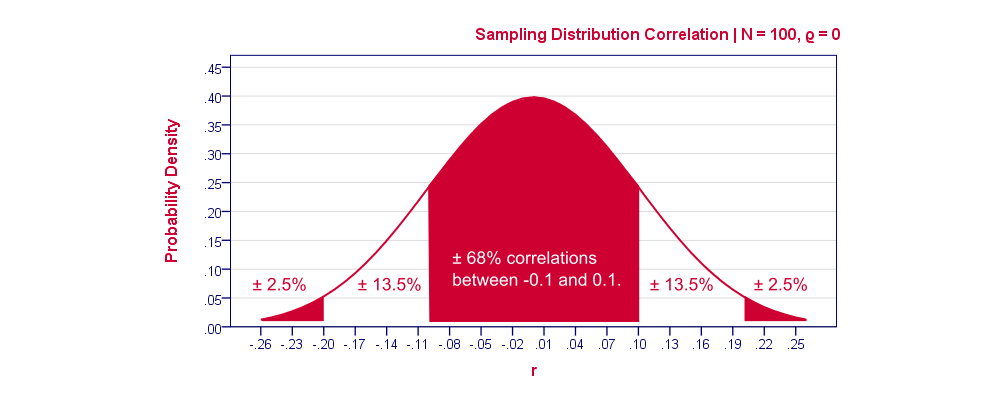Source

For example, after collect 100 data points, we can calculate a correlation coefficient based on the data. As shown above, if the correlation is -0.25 for the 100 data points we collected, its corresponding PDF is about 0.012. Only 2.5% of the population may have correlation smaller than -0.2. Therefore, the null hypothesis is likely false.

Confidence interval

After conducting an experiment to collect a sample. We can use the sample data points to estimate a population parameter like the mean (called estimator). A confidence interval can be calculated as a range around this sample mean. A 95% confidence level means that in 95% of those experiments, its confidence interval contains the true mean of the population. In other words, 1 out of 20 chance that the confidence interval of an experiment does not contain the true mean.

Here is the skeleton in calculating the confidence interval for the sample mean.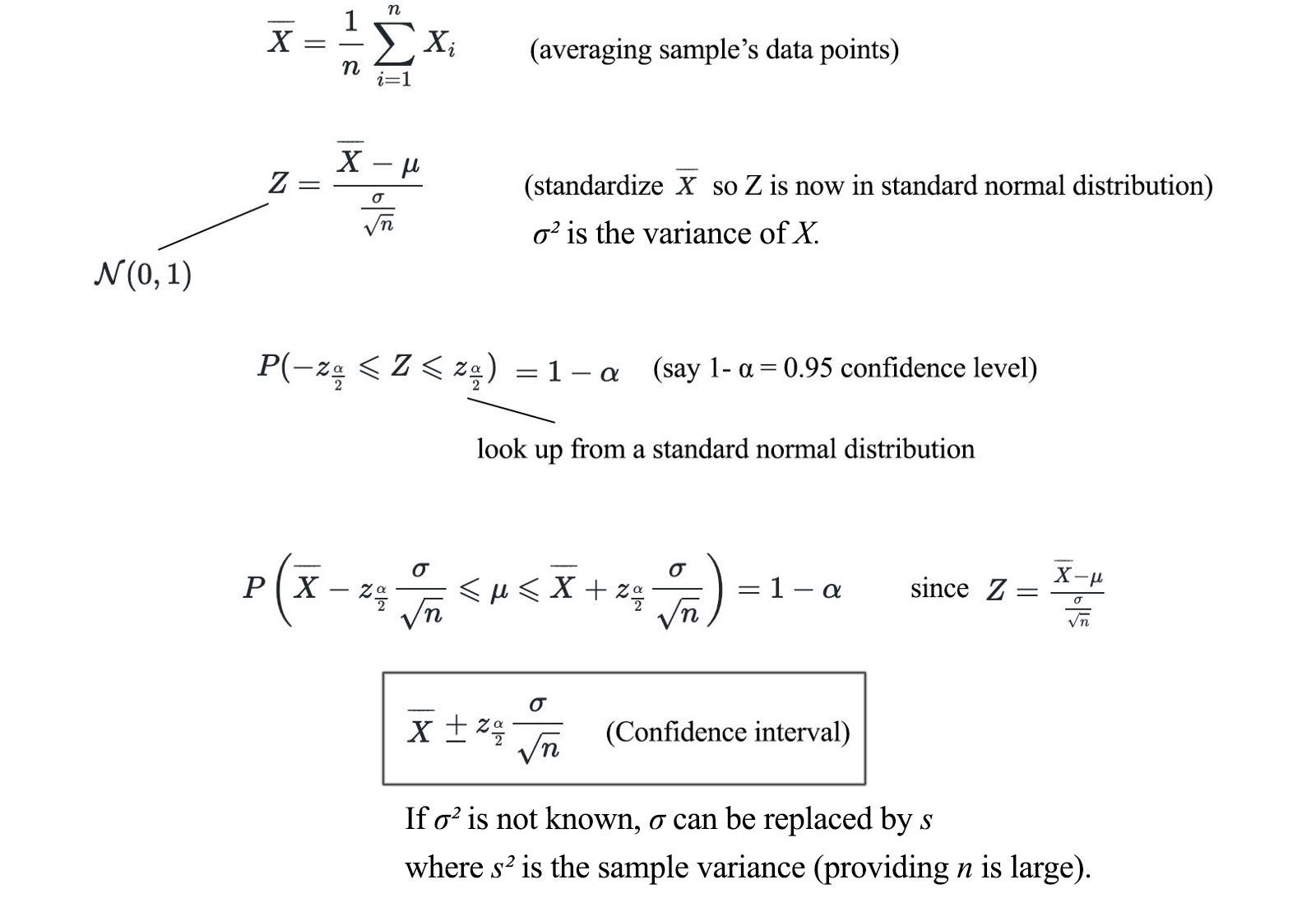Modified from source

And the sample variance: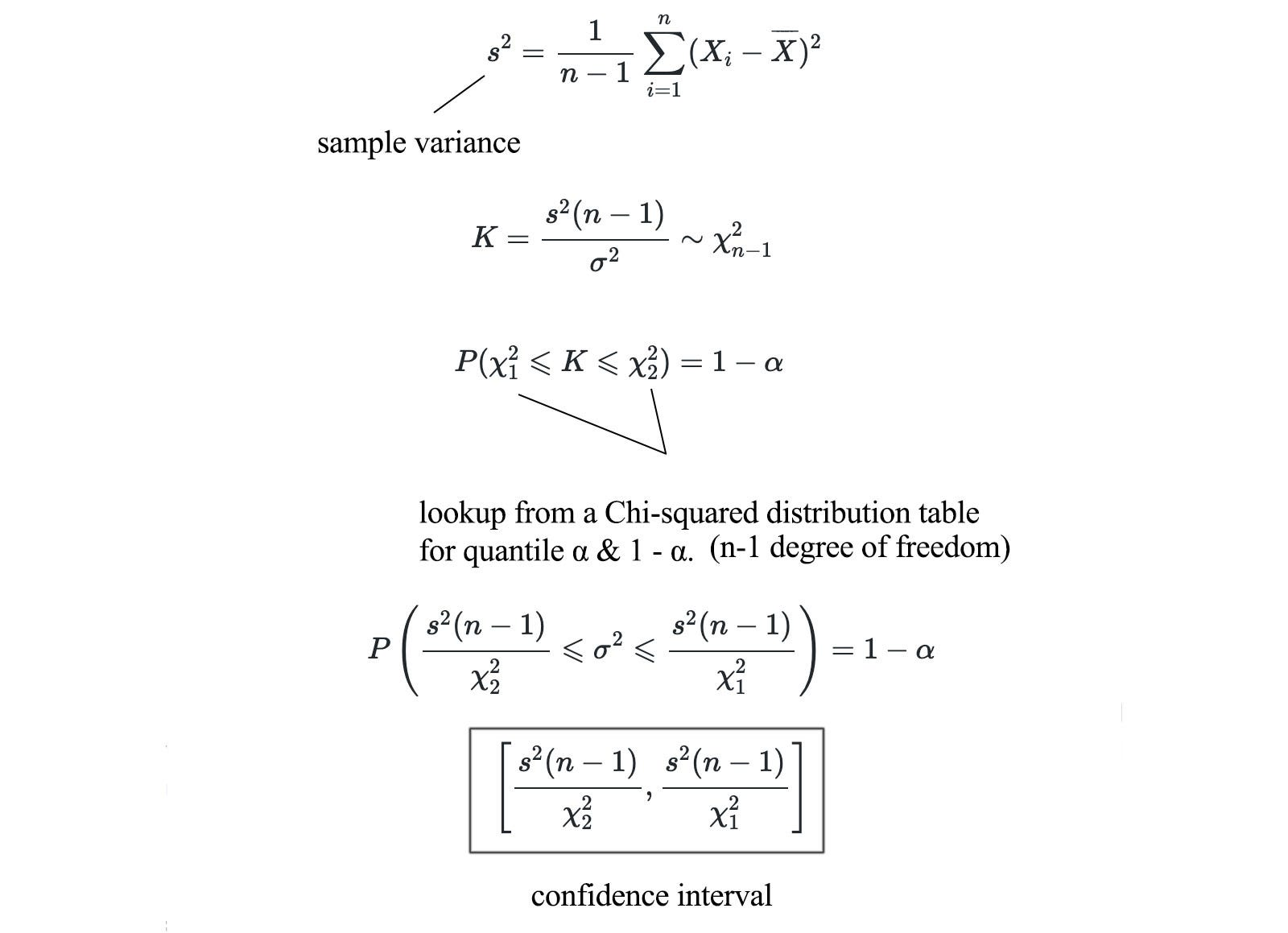Modified from source

Chi-square test

Chi-square test is a popular test in measuring the likelihood that the correlation in the observed data is by chance only, rather than some correlation between two variables.

We compute the Chi-square value with the formula above. We compare the real count from the sample and the expected count assuming no correlation exists. Start from the Chi-square, we can look up the corresponding p-value from a pre-computed table. If the Chi-square coefficient is close to zero, H₀ is true. Here is an example below in determining whether gender impacts the choice of a pet.

In this example, the p-value corresponding to the calculated Chi-square coefficient is small. Therefore, gender and choice of pet are related.

### Exploratory data analysis

To explore the data, we may calculate the covariance between two variables or perform a scatter plot like the one below to spot trends.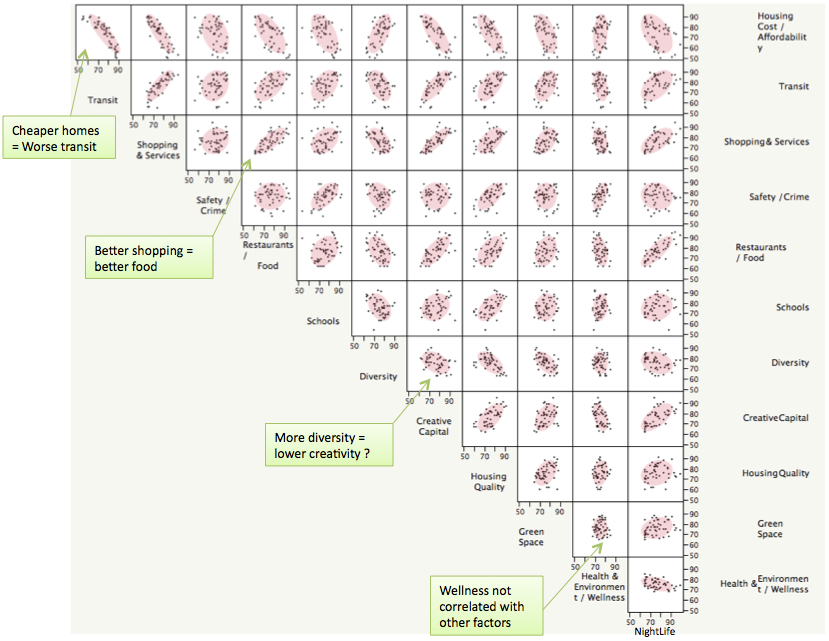Source

For example, the green dots and the blue dots below are houses for SF and NY respectively. If we have a decision stump for elevation > 73 feet, the one satisfies this condition will likely in SF.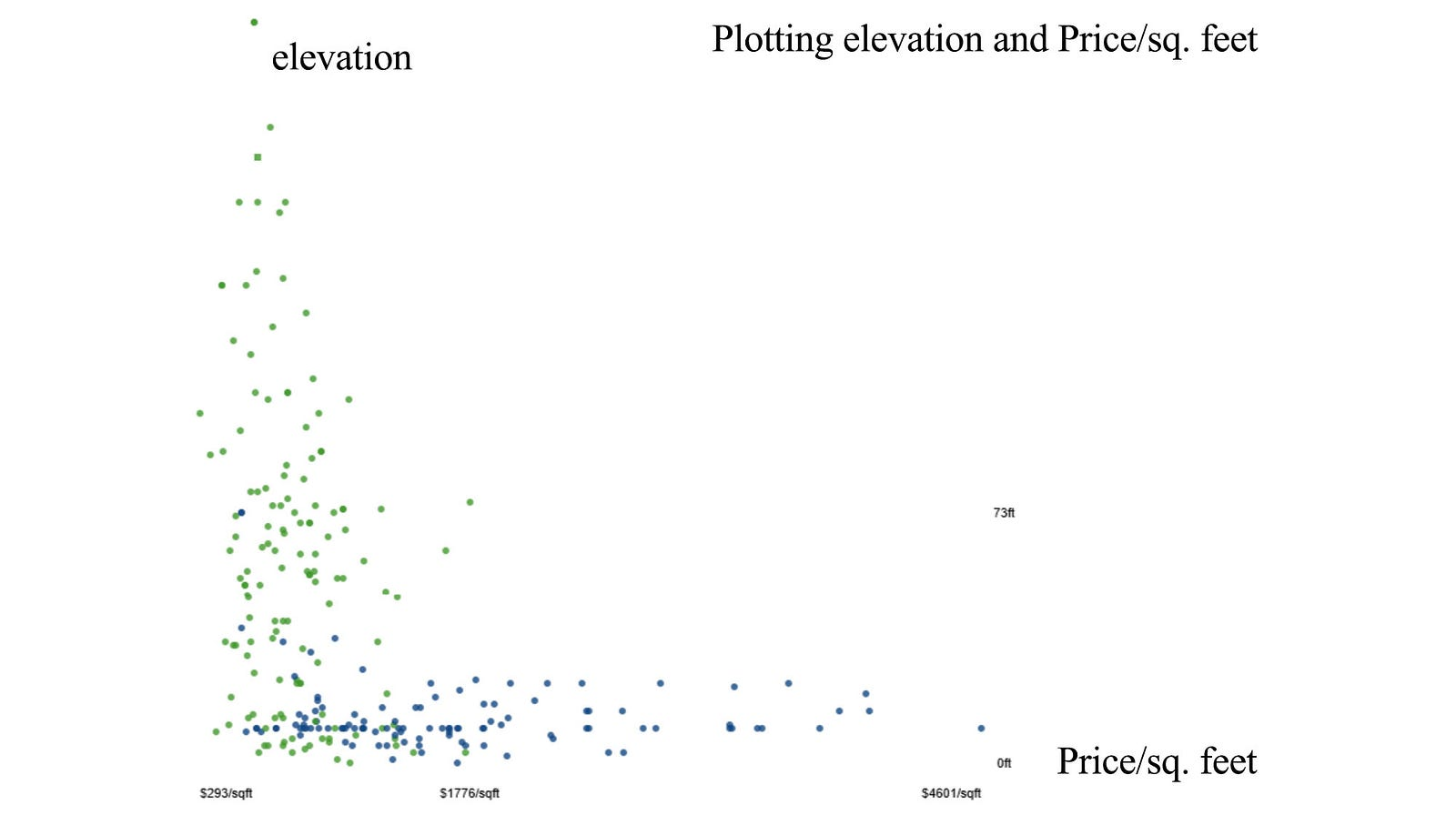Modified from source (but the data can be outdated)

### Norms

L1, L2-norm

Lp-norm, L∞-norm (max norm) & Frobenius norm

### Similarity

Jaccard Similarity

The Jaccard similarity measures the ratio between the size of the intersection over the size of the union.

Cosine Similarity

Cosine similarity measures the angle between two vectors.

Pearson Similarity

Pearson correlation coefficient ρ measures the correlation between two variables.

### Courses

Here are some free courses in ML.

Andrew Ng Course

John W. Paisley Course

Nando de Freitas Course

Mark Schmidt Course

### Next

Each section in the article can be spawned off to a lengthy article. So congratulation that you make it so far. Next, we will dig into more traditional ML algorithms.